# Selina Solutions Concise Maths Class 10 Chapter 14 Equation of a Line

This Chapter is one of the important chapters of Class 10 ICSE. The topics covered under this Chapter are basic concept of a straight line equation, inclination of a line, concept of slope (gradient), slope of a straight line passing through two given fixed points, slopes of parallel and straight lines, various forms of the equations of straight lines and finding x/y -intercepts of a given line. Also, creating a good understanding of these concepts lays a strong foundation in higher classes. Students wishing to get a good grip over the chapters of Selina Solutions for Class 10 Mathematics can download the PDF of solutions prepared by subject experts at BYJU’S. Students can now access the Selina Solutions for Class 10 Mathematics Chapter 14 Equation of a Line from the links enclosed below.

## Selina Solutions Concise Maths Class 10 Chapter 14 Equation of a Line Download PDF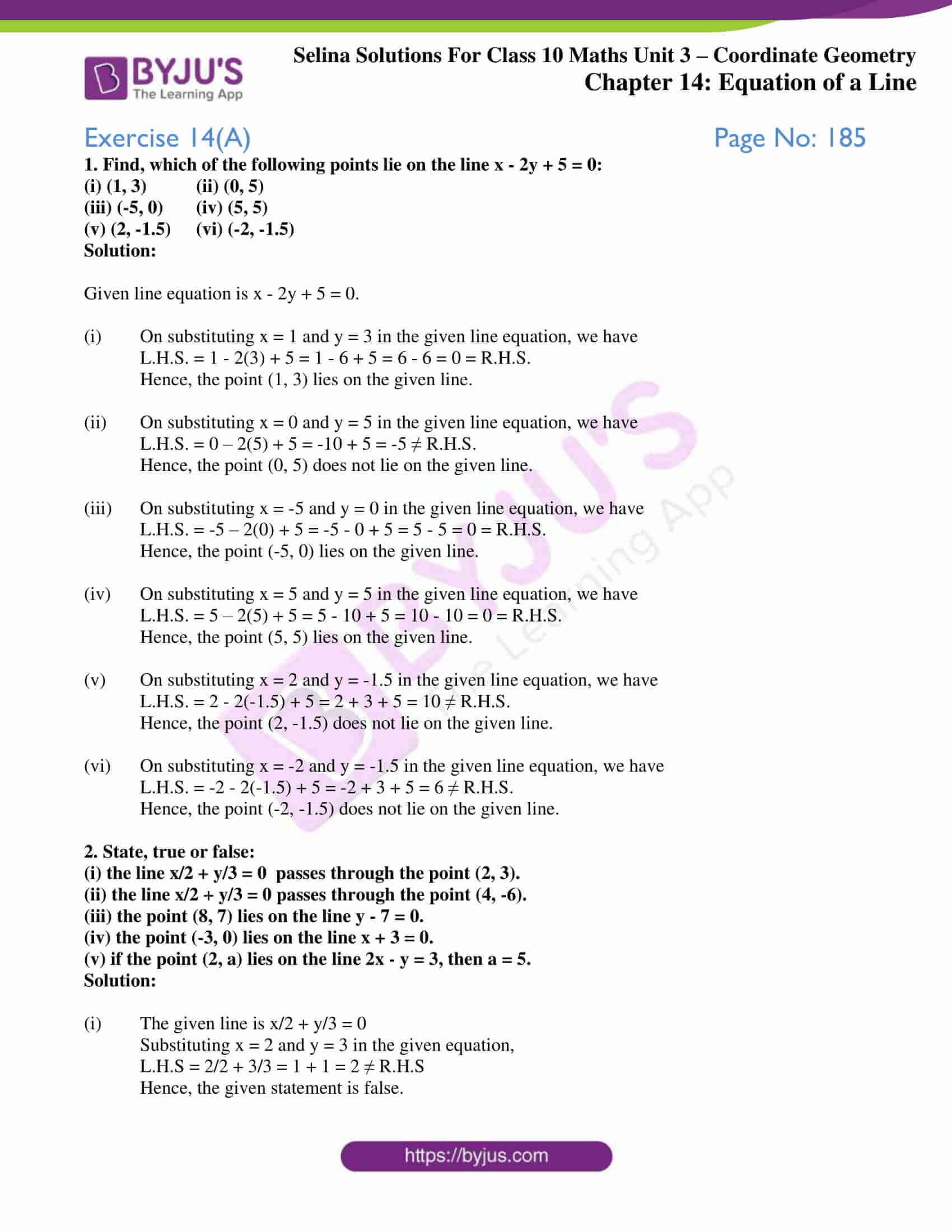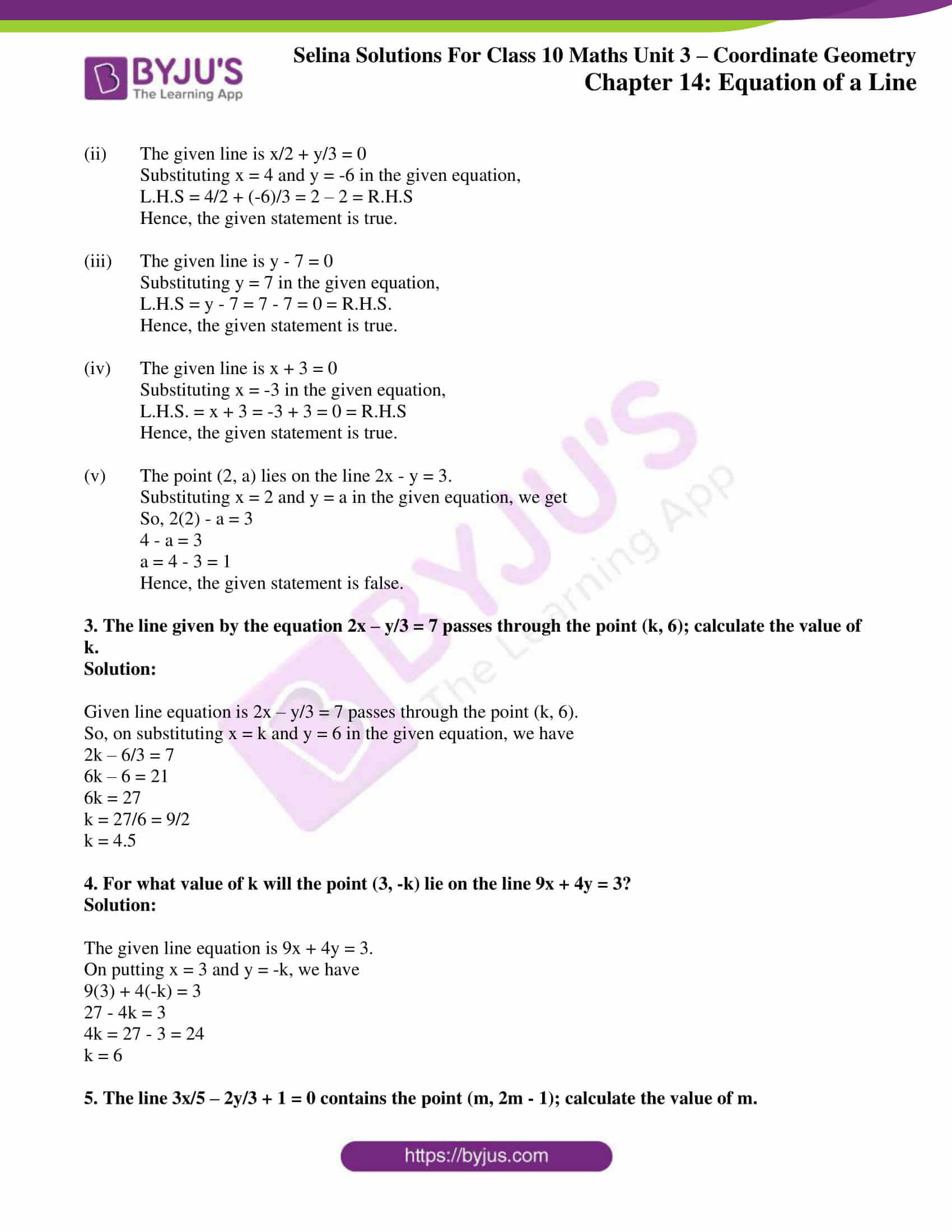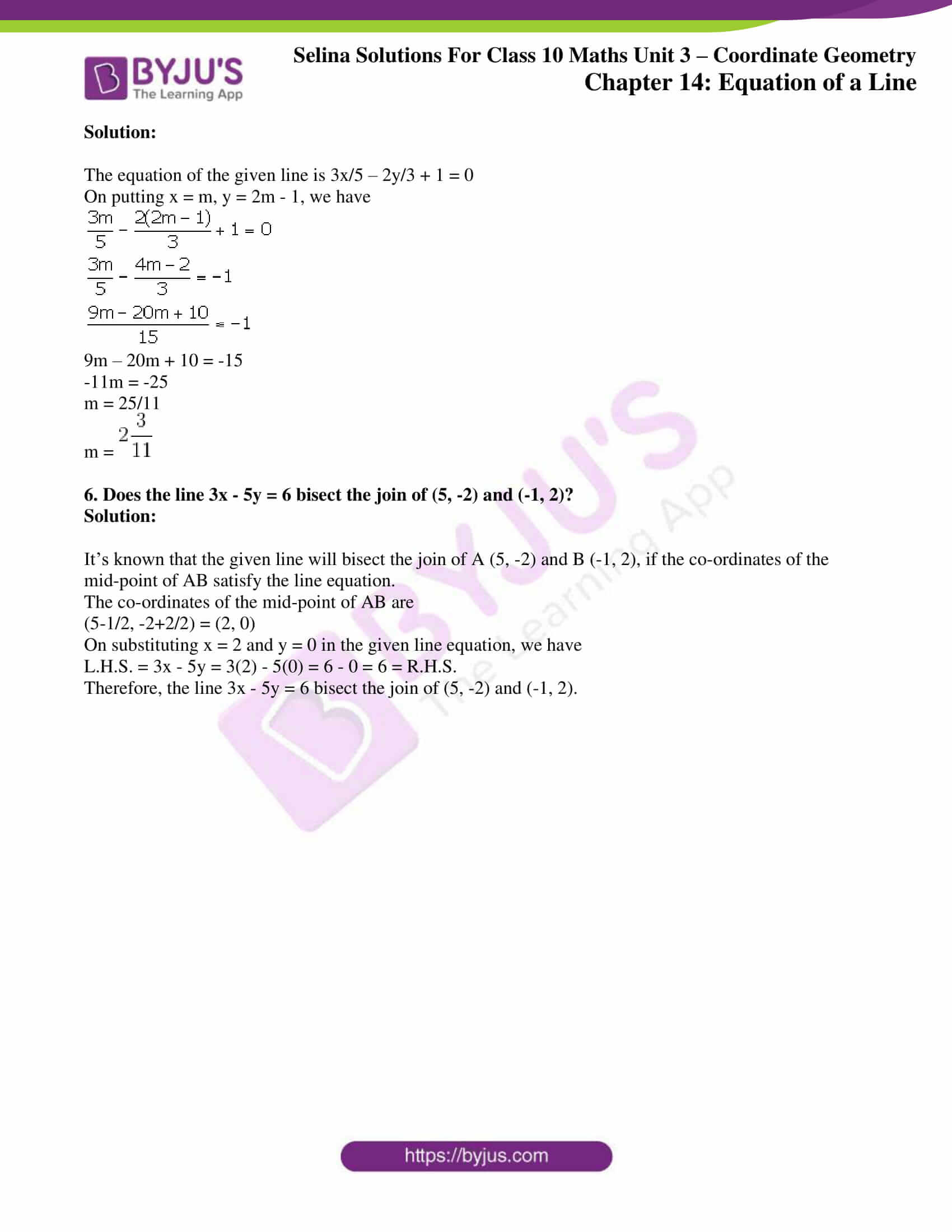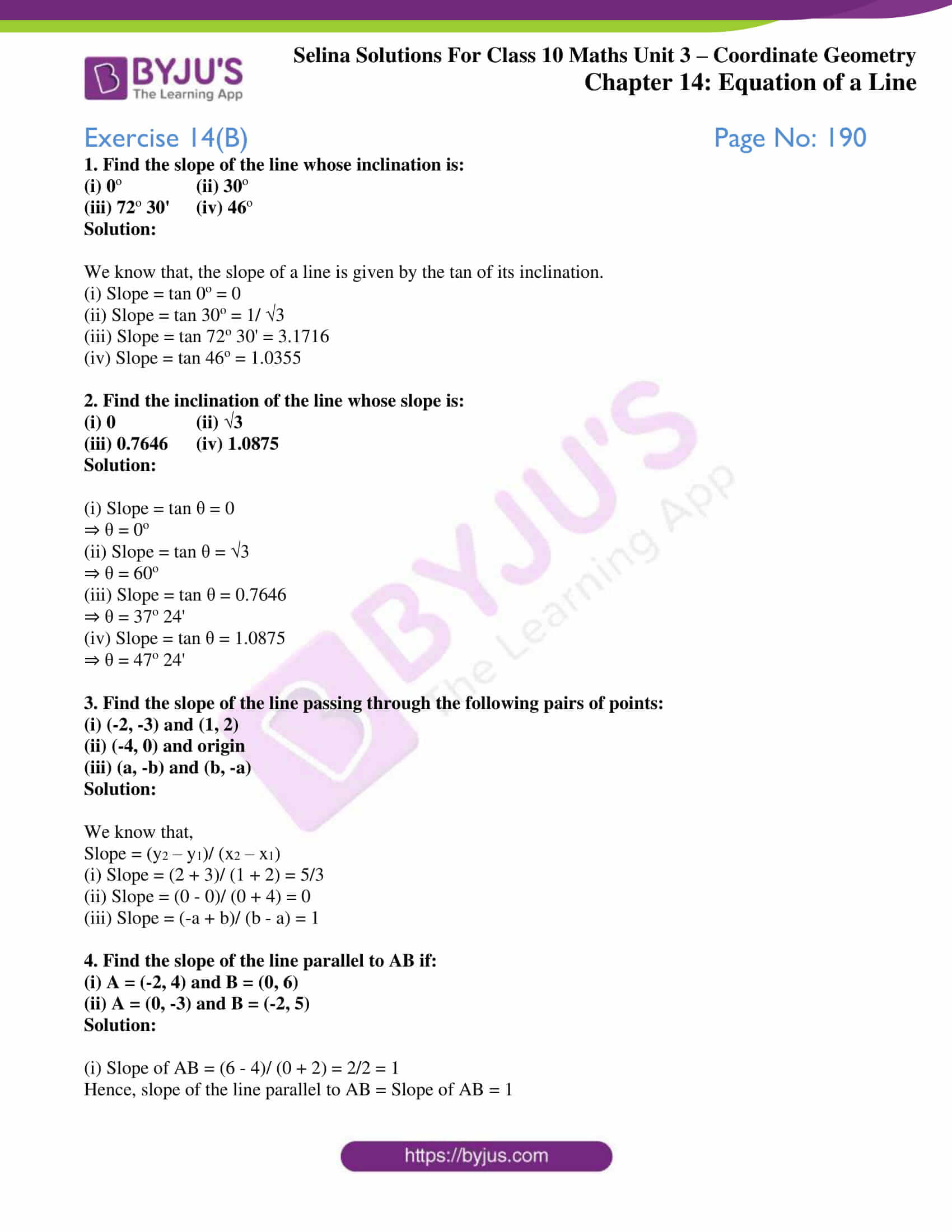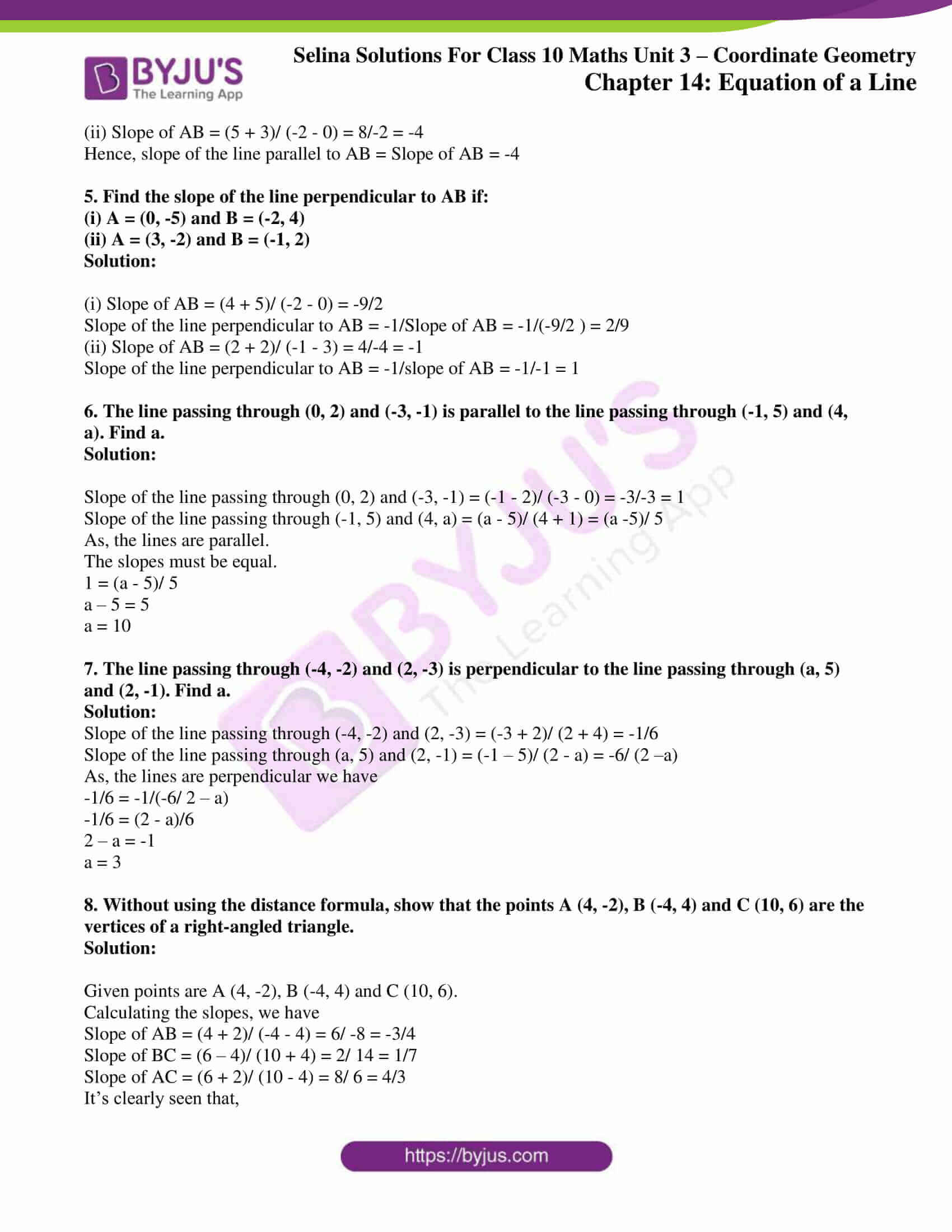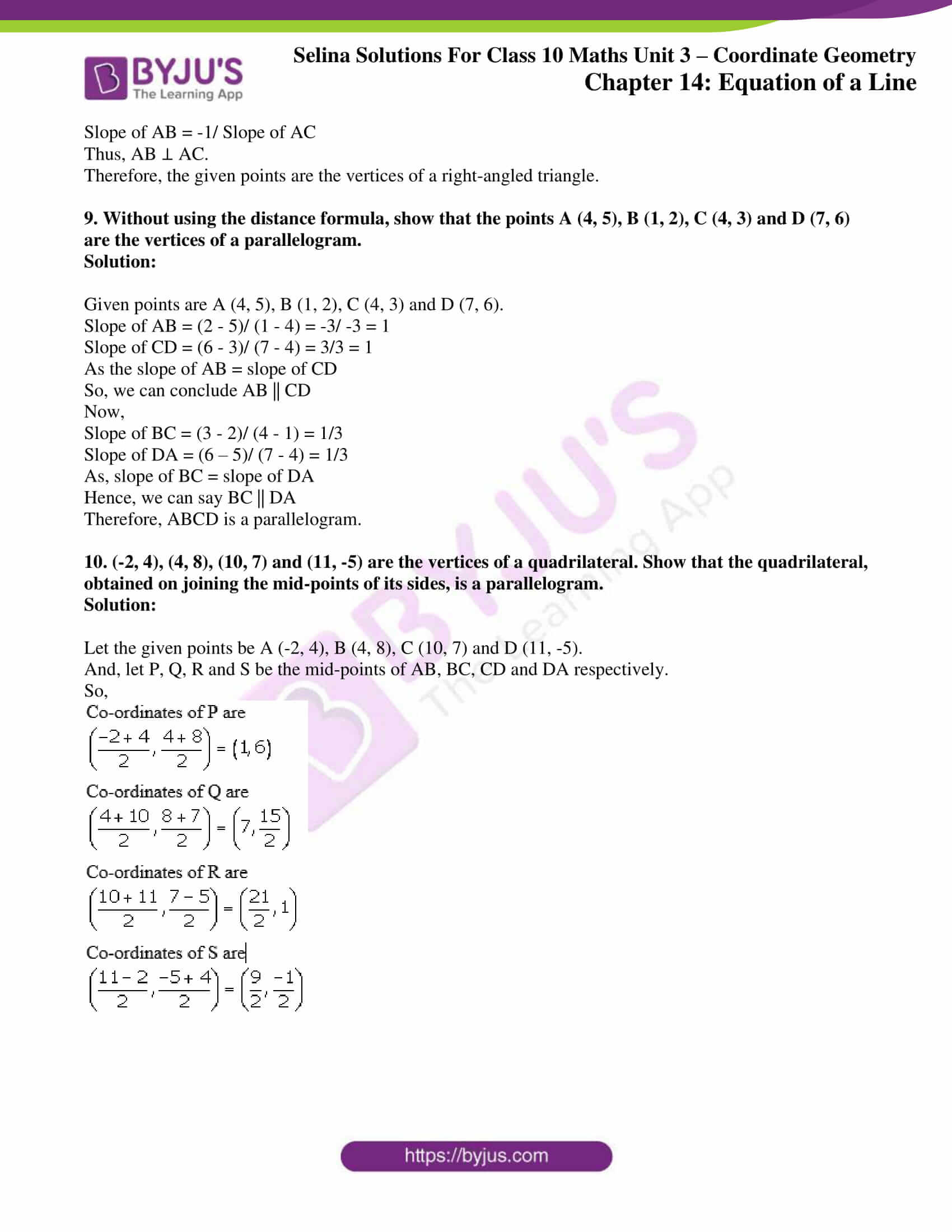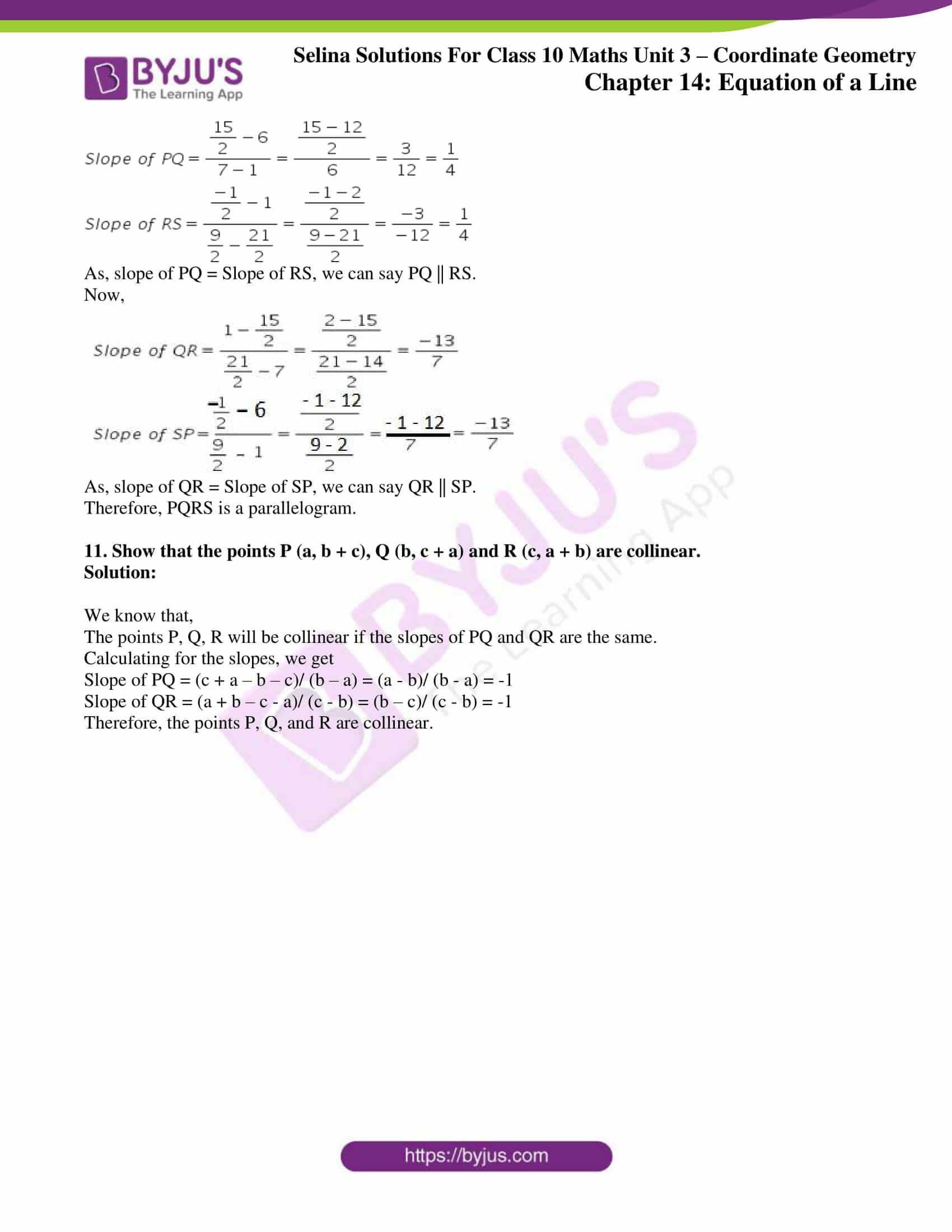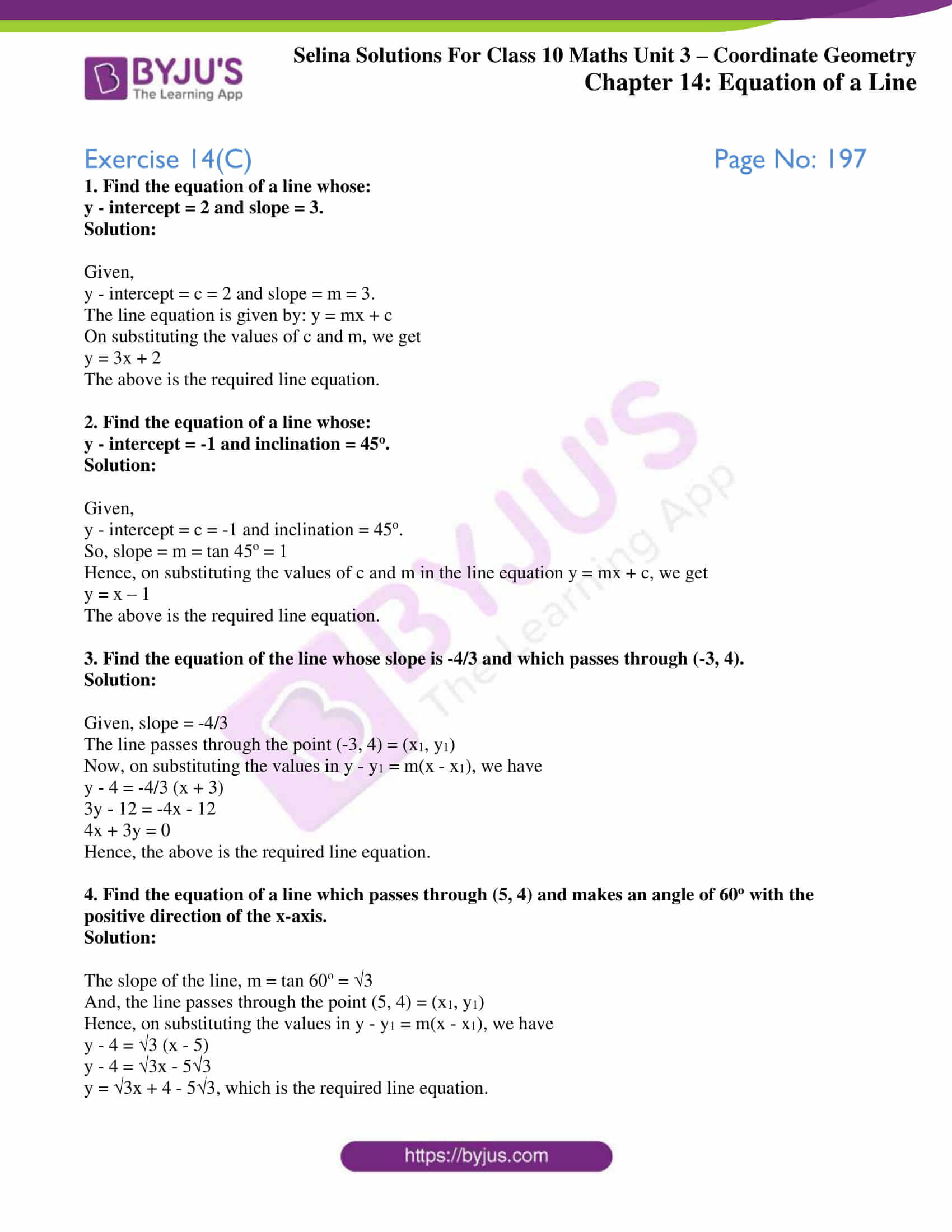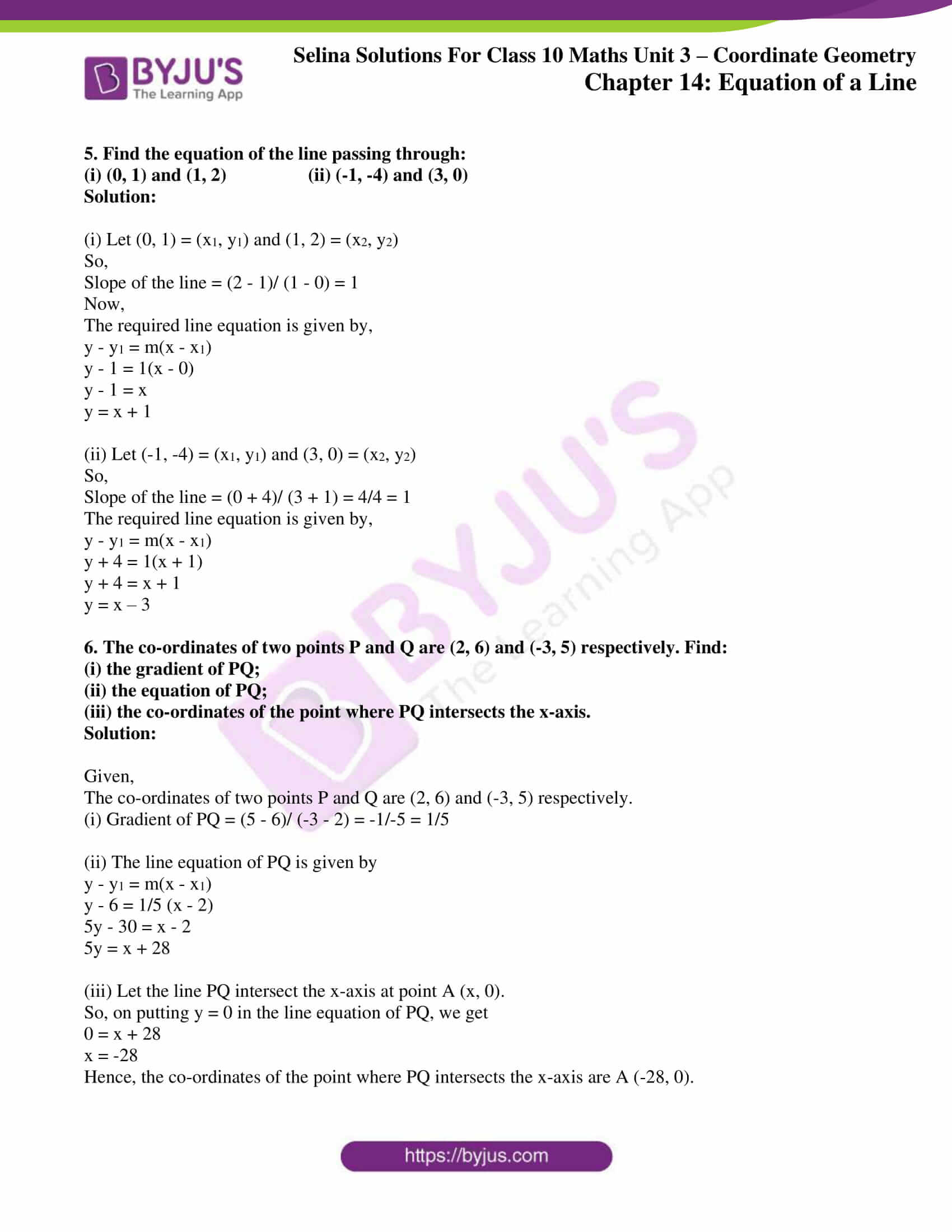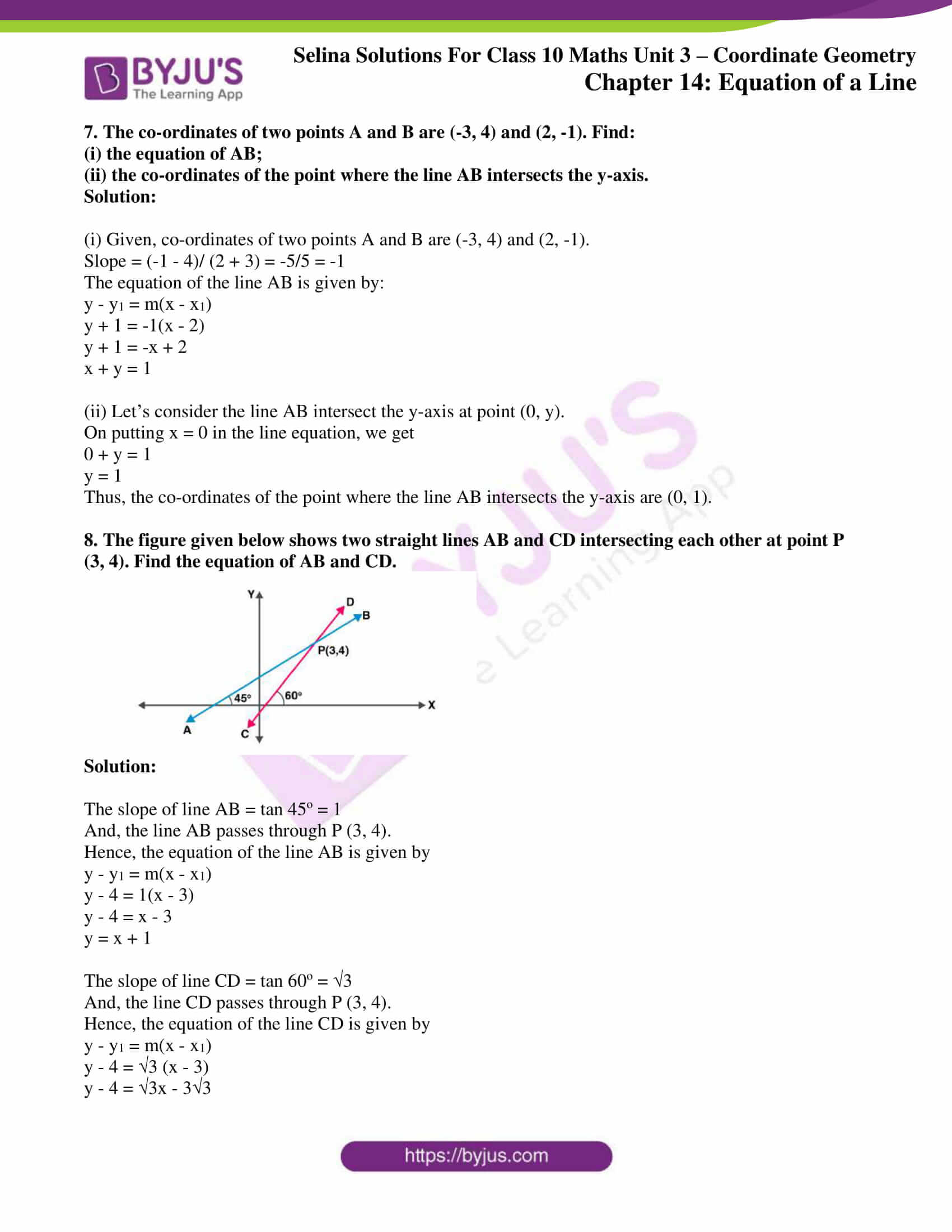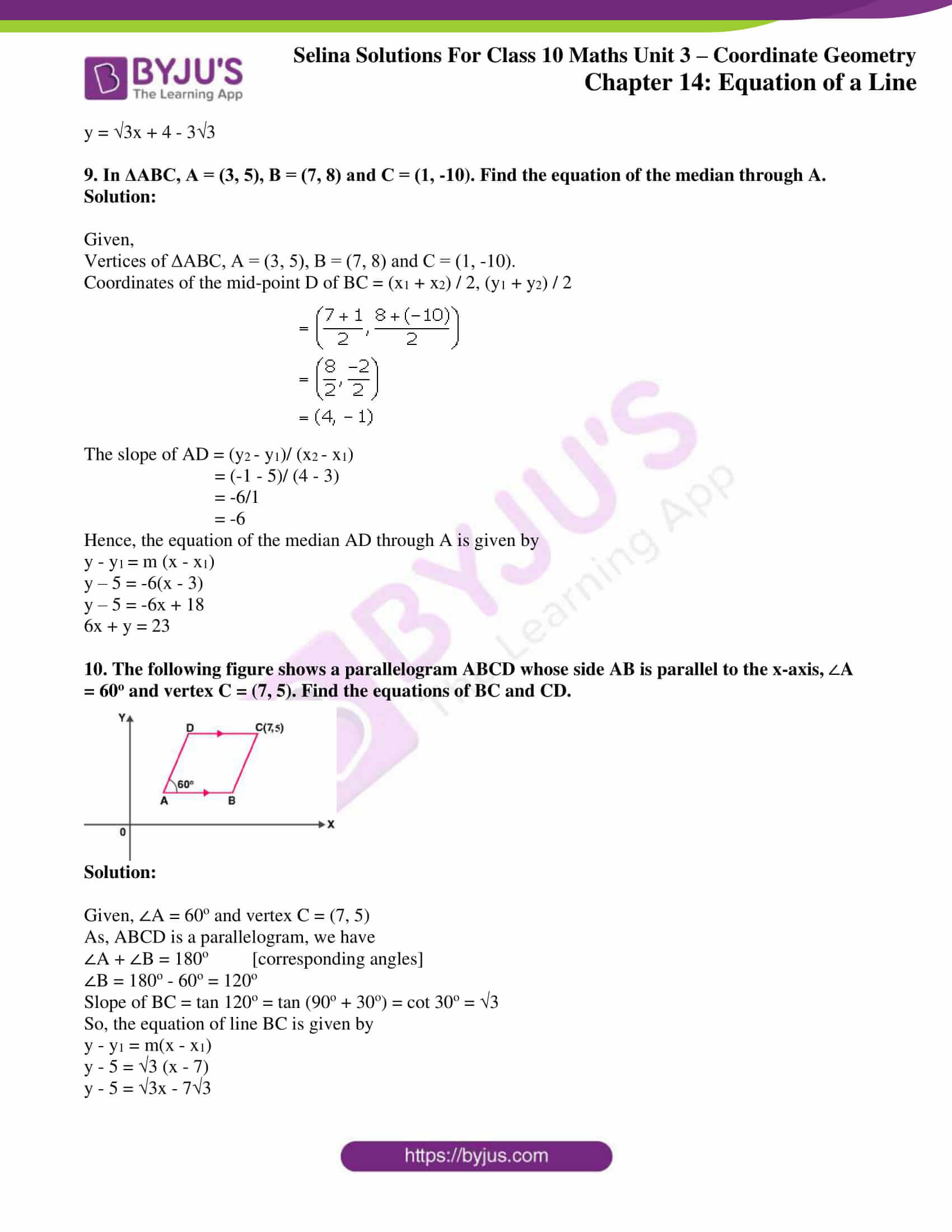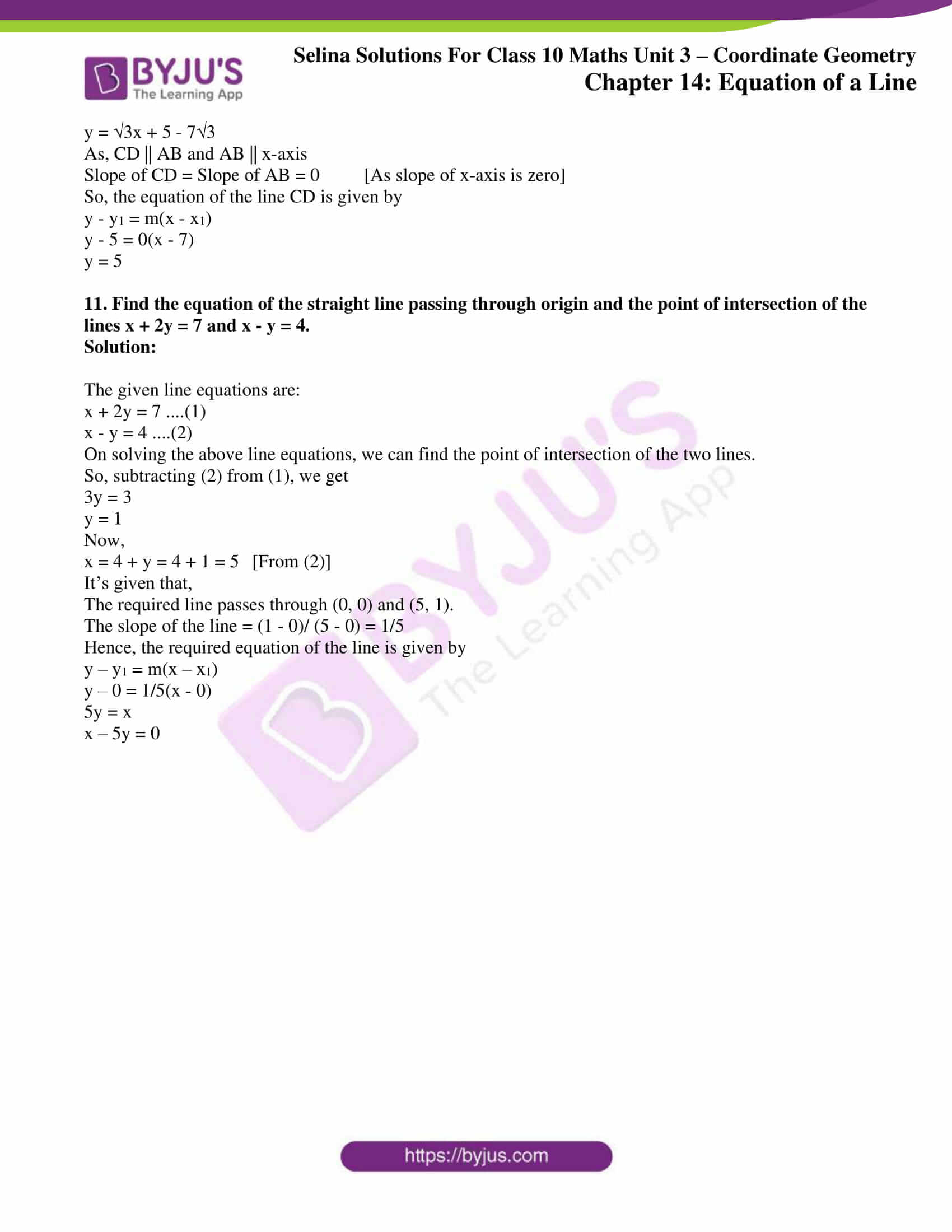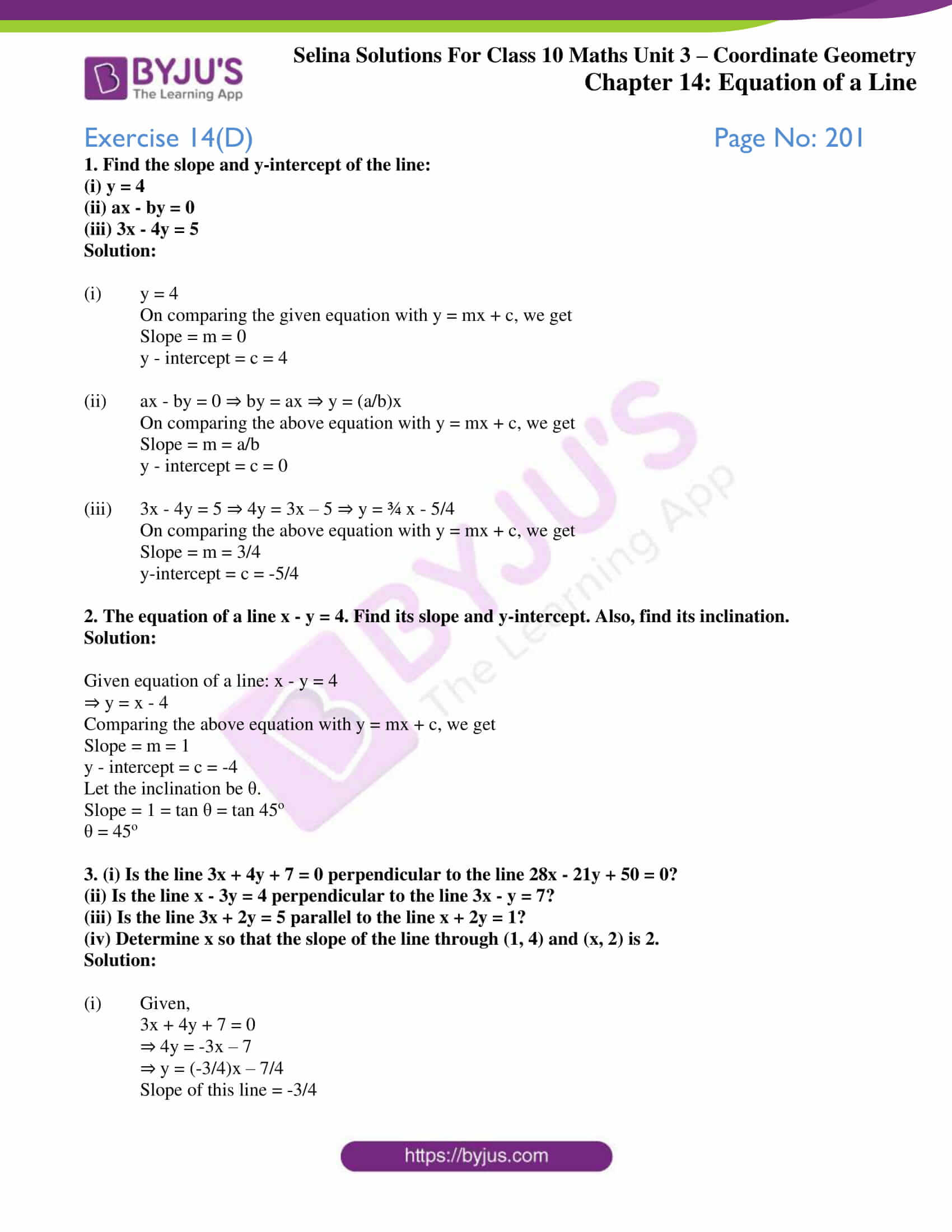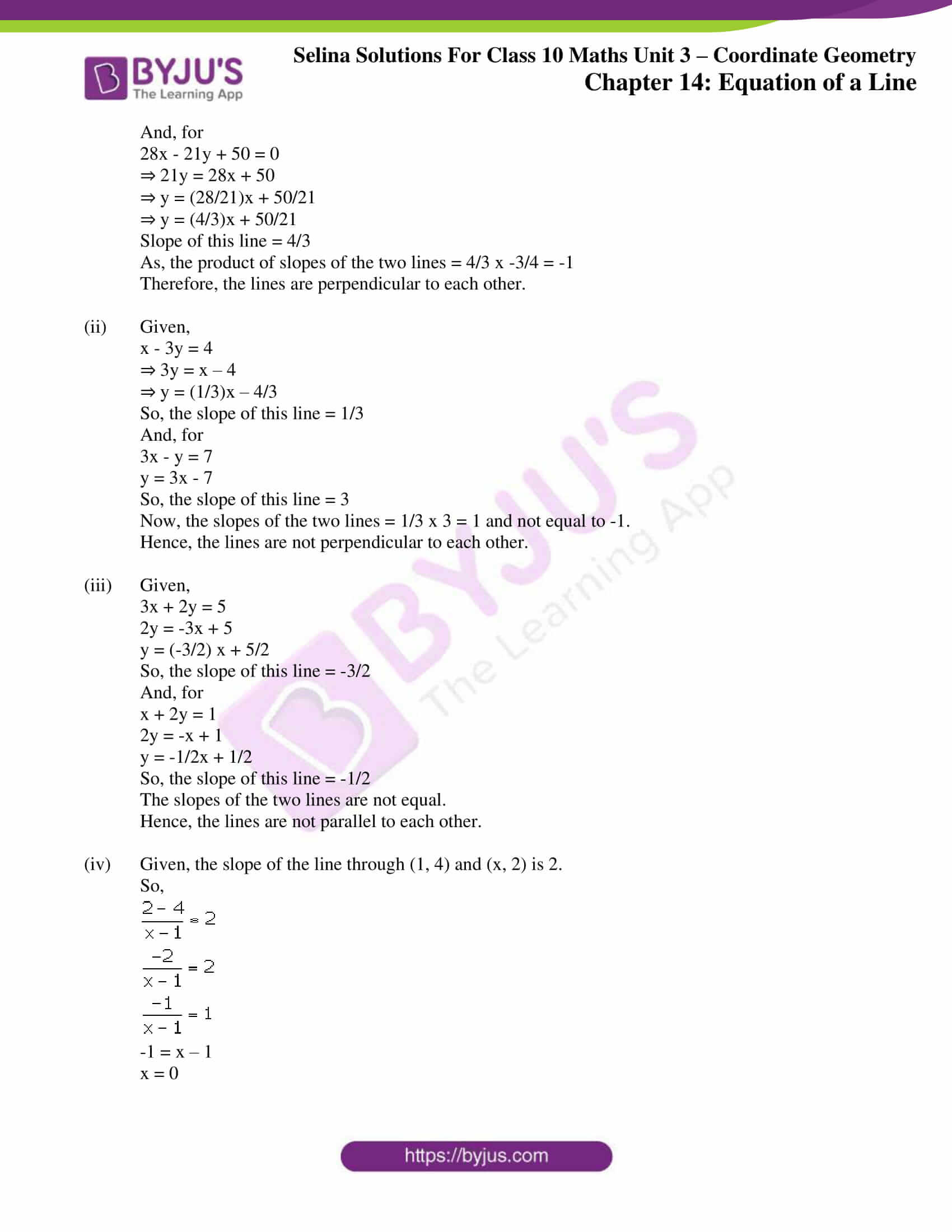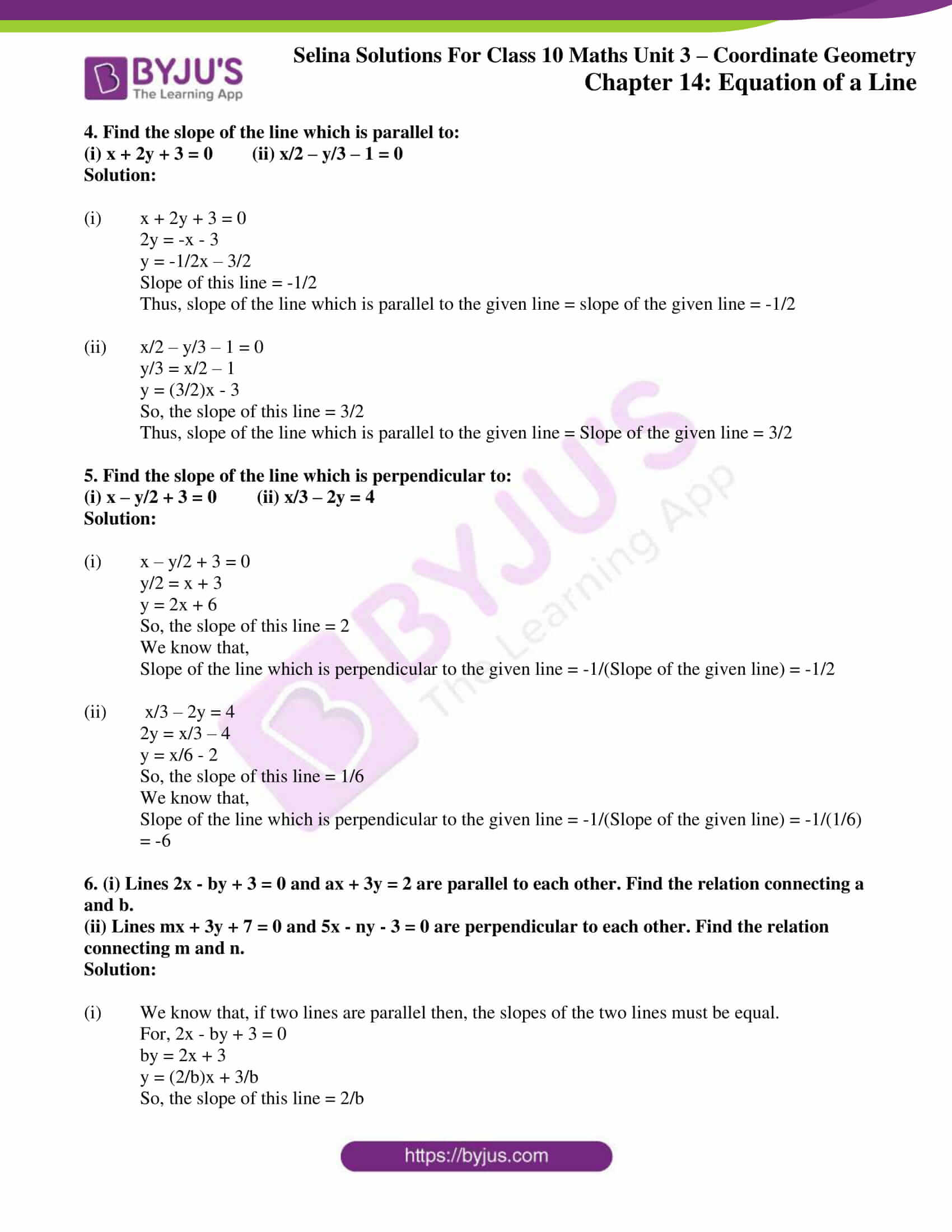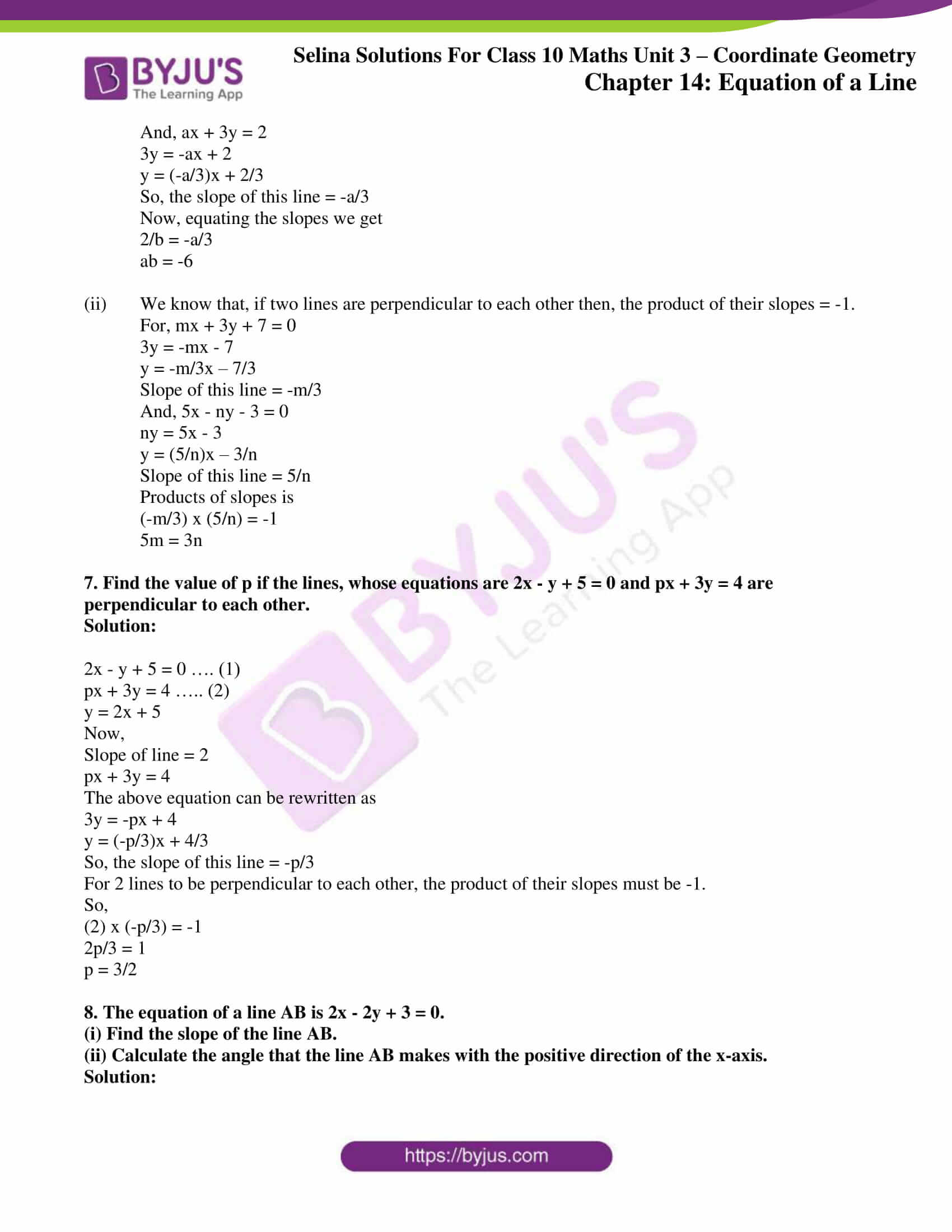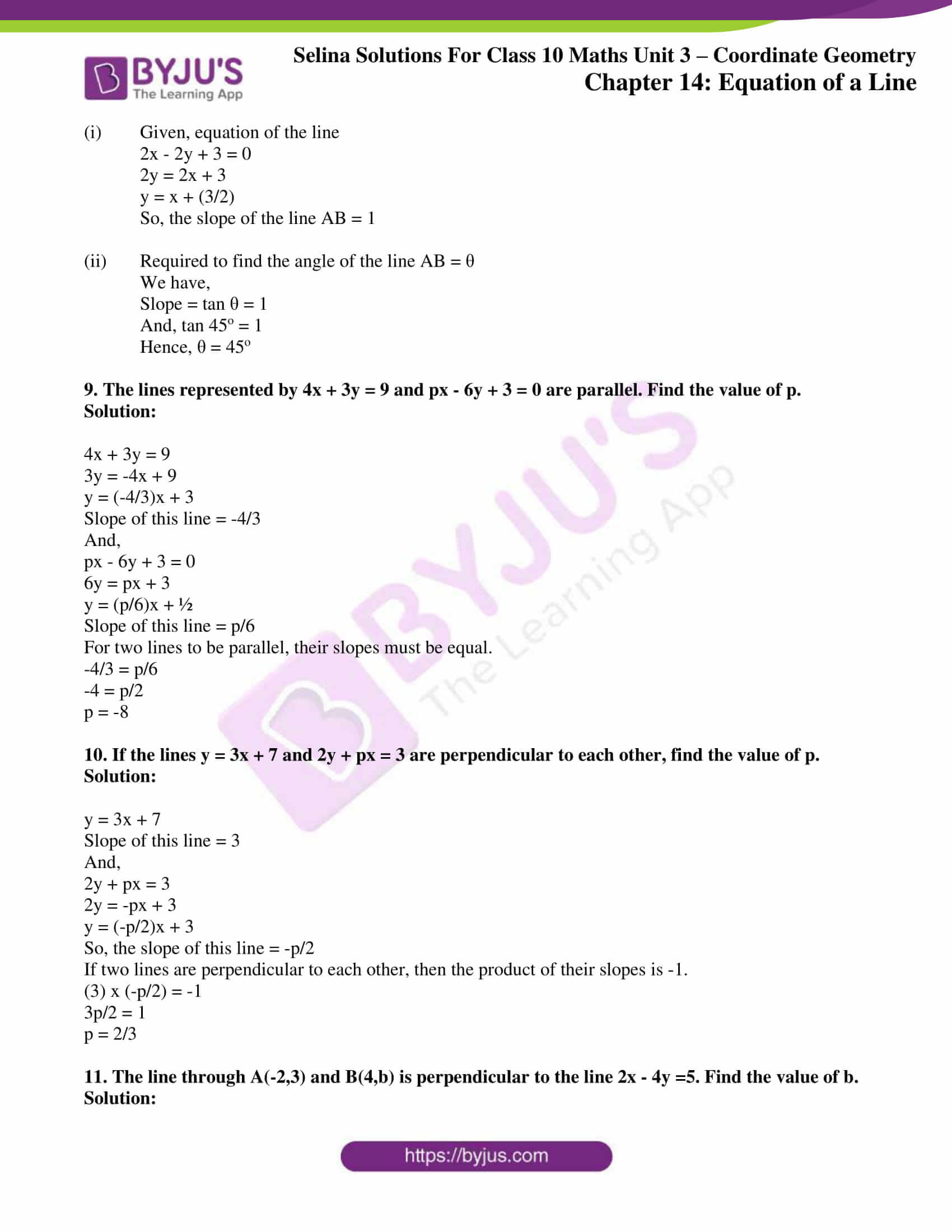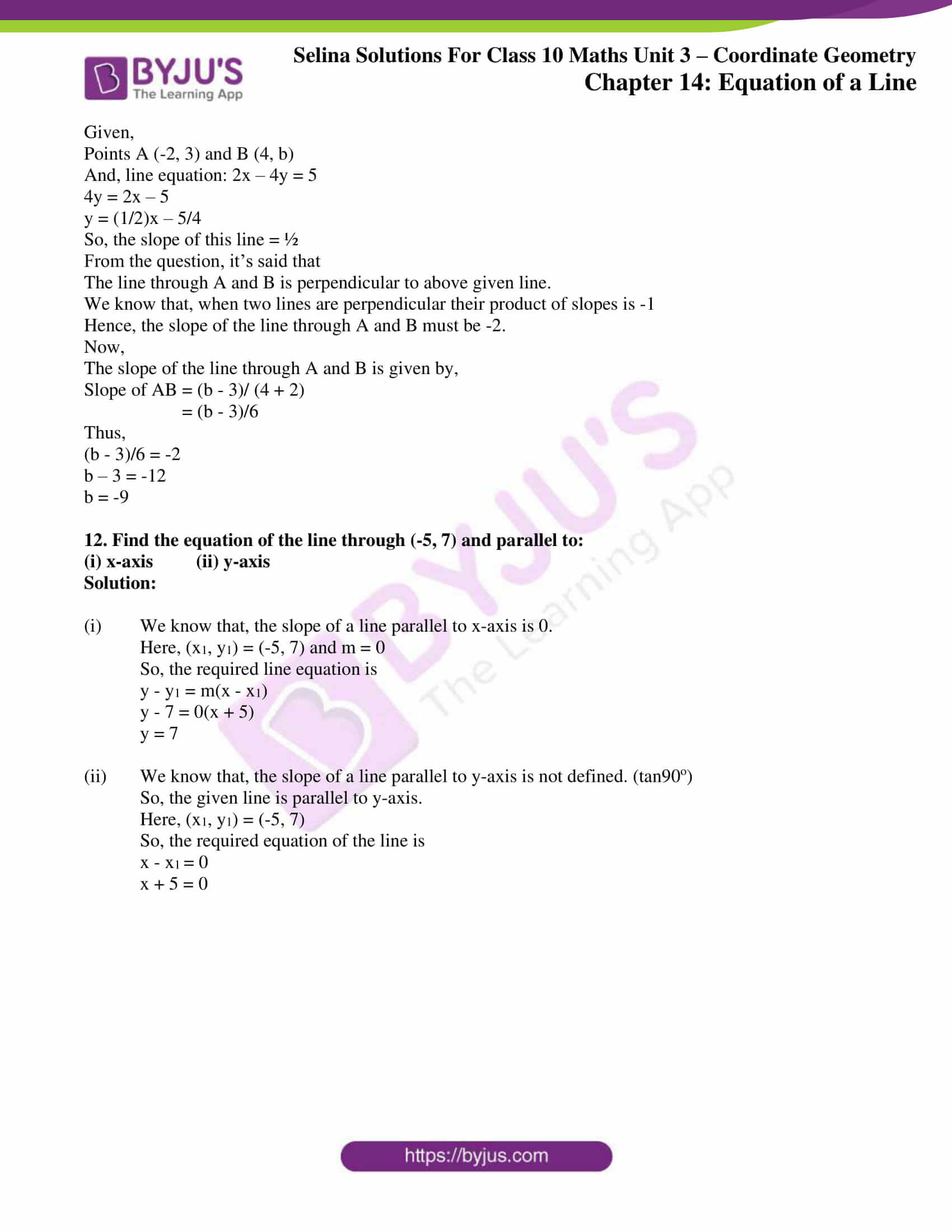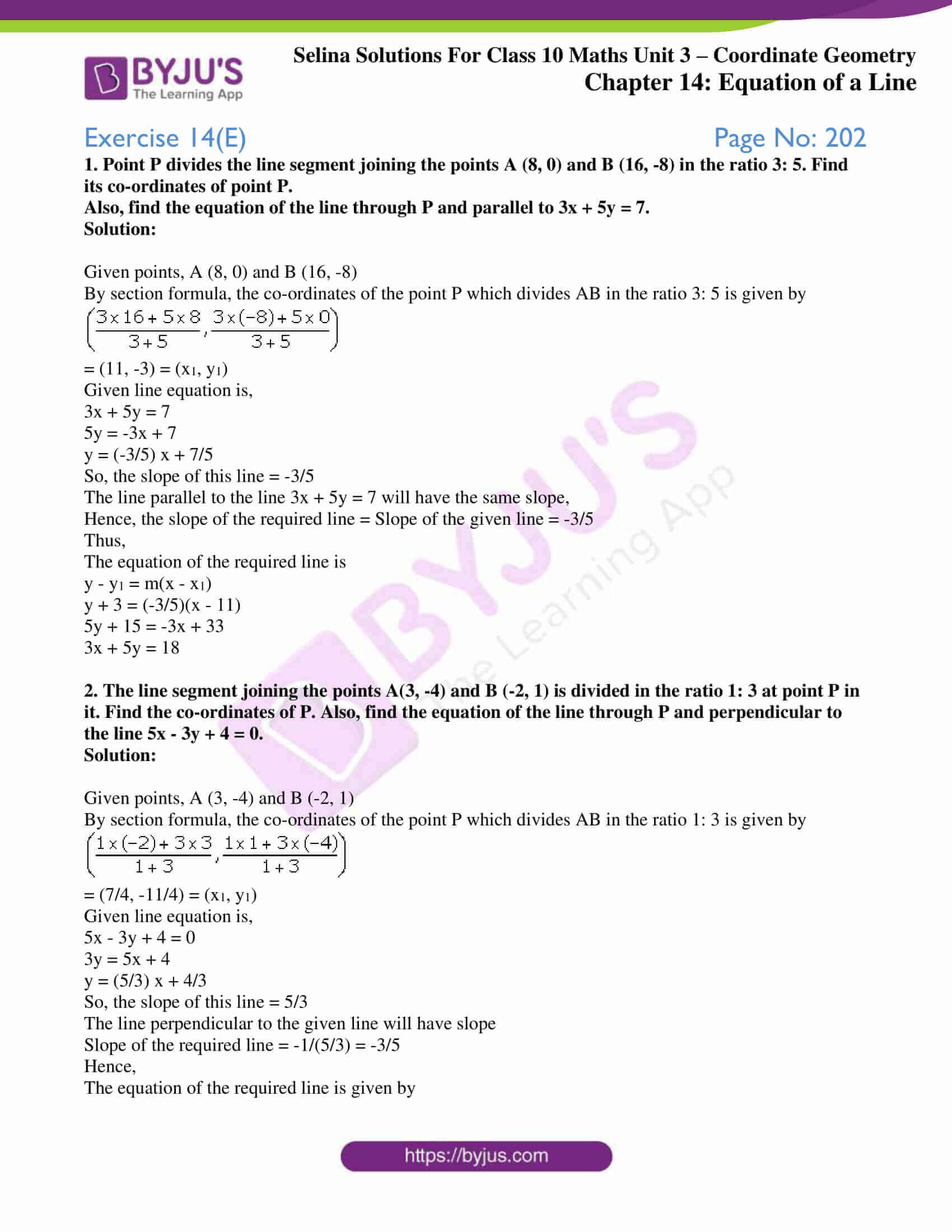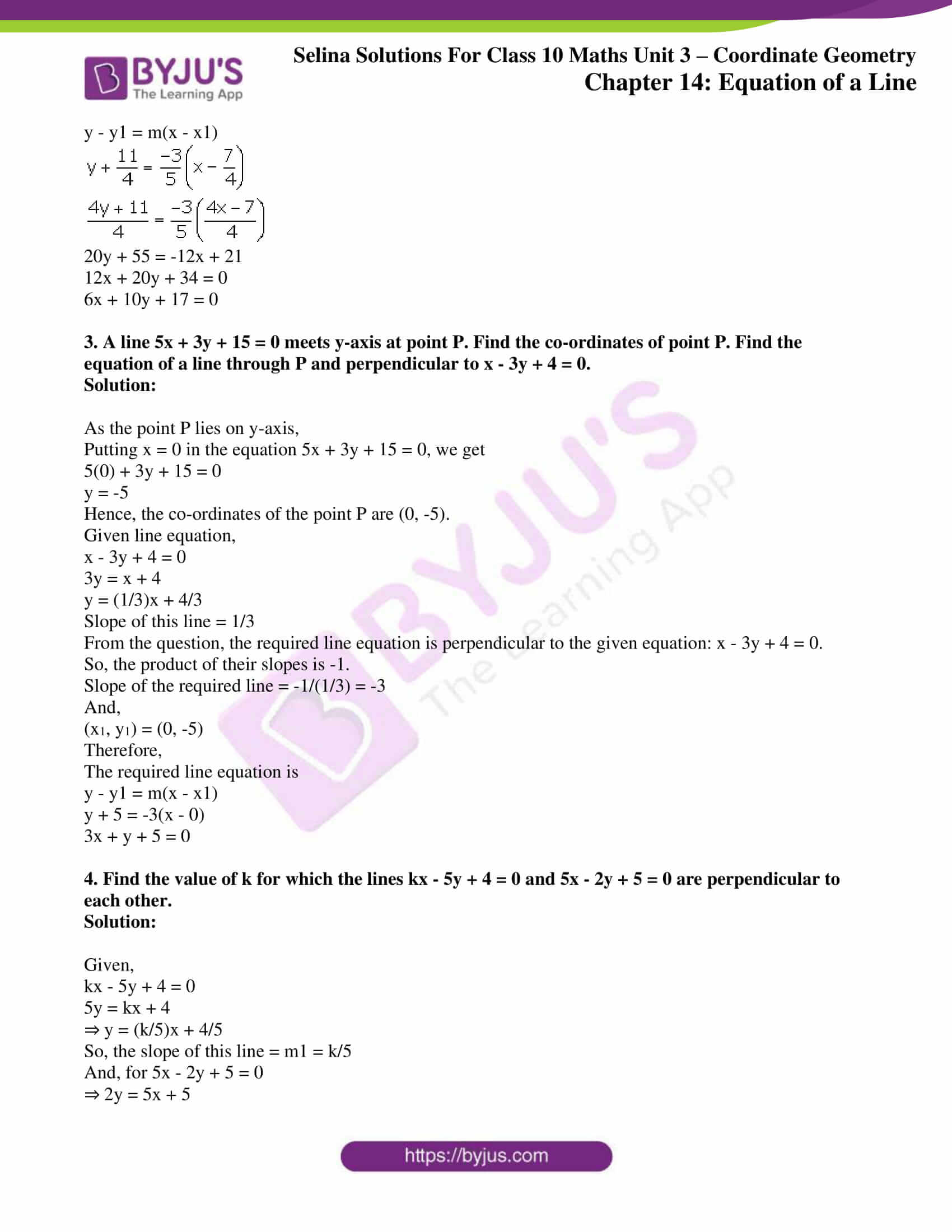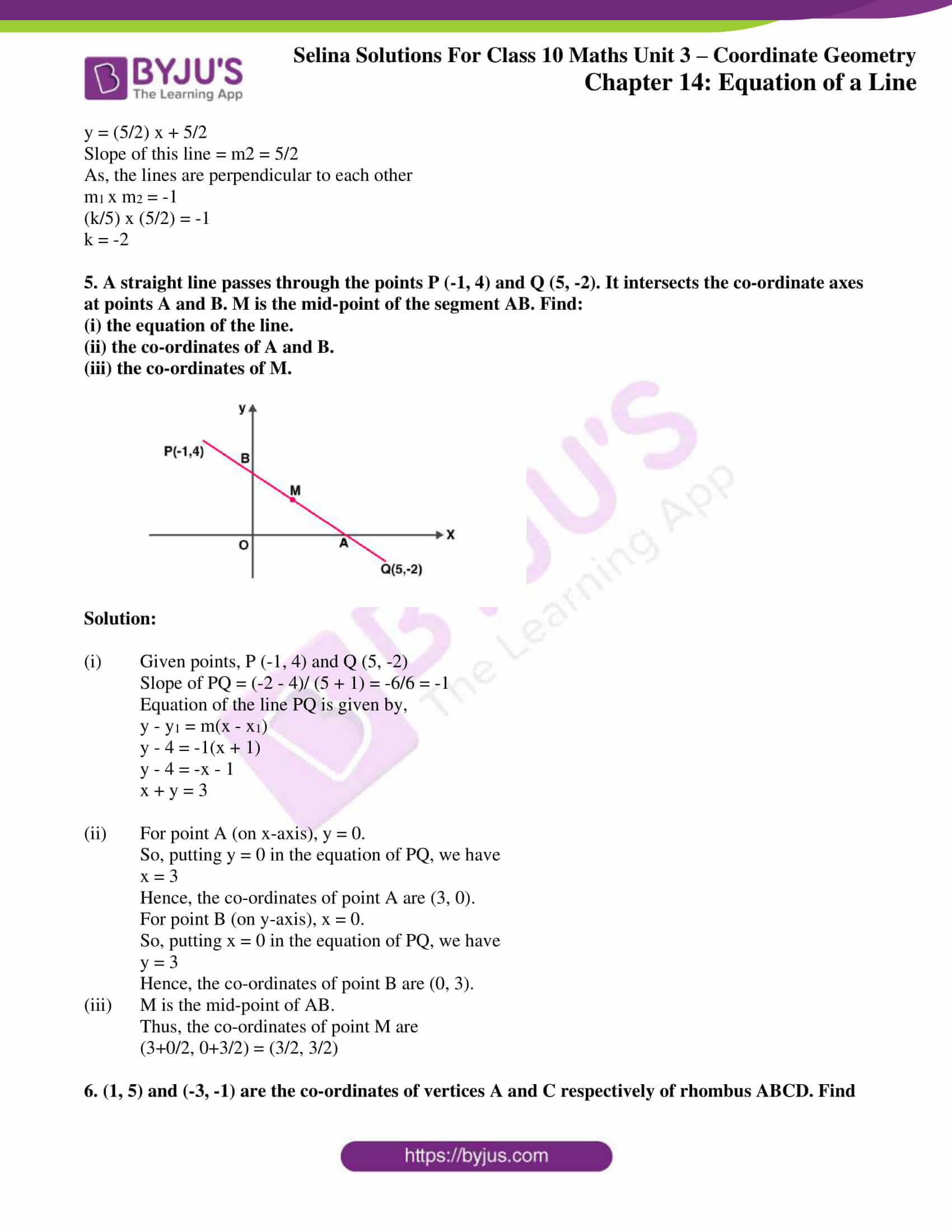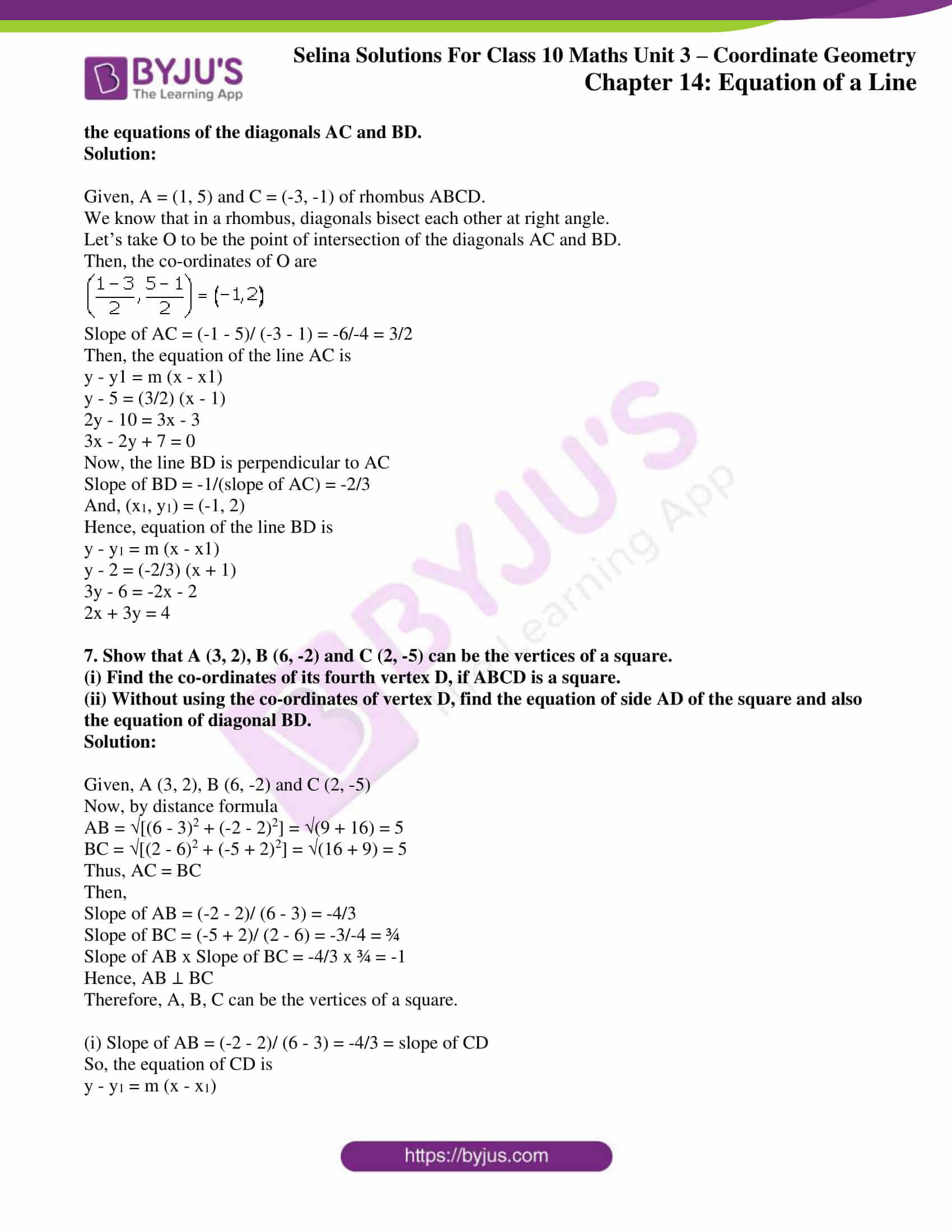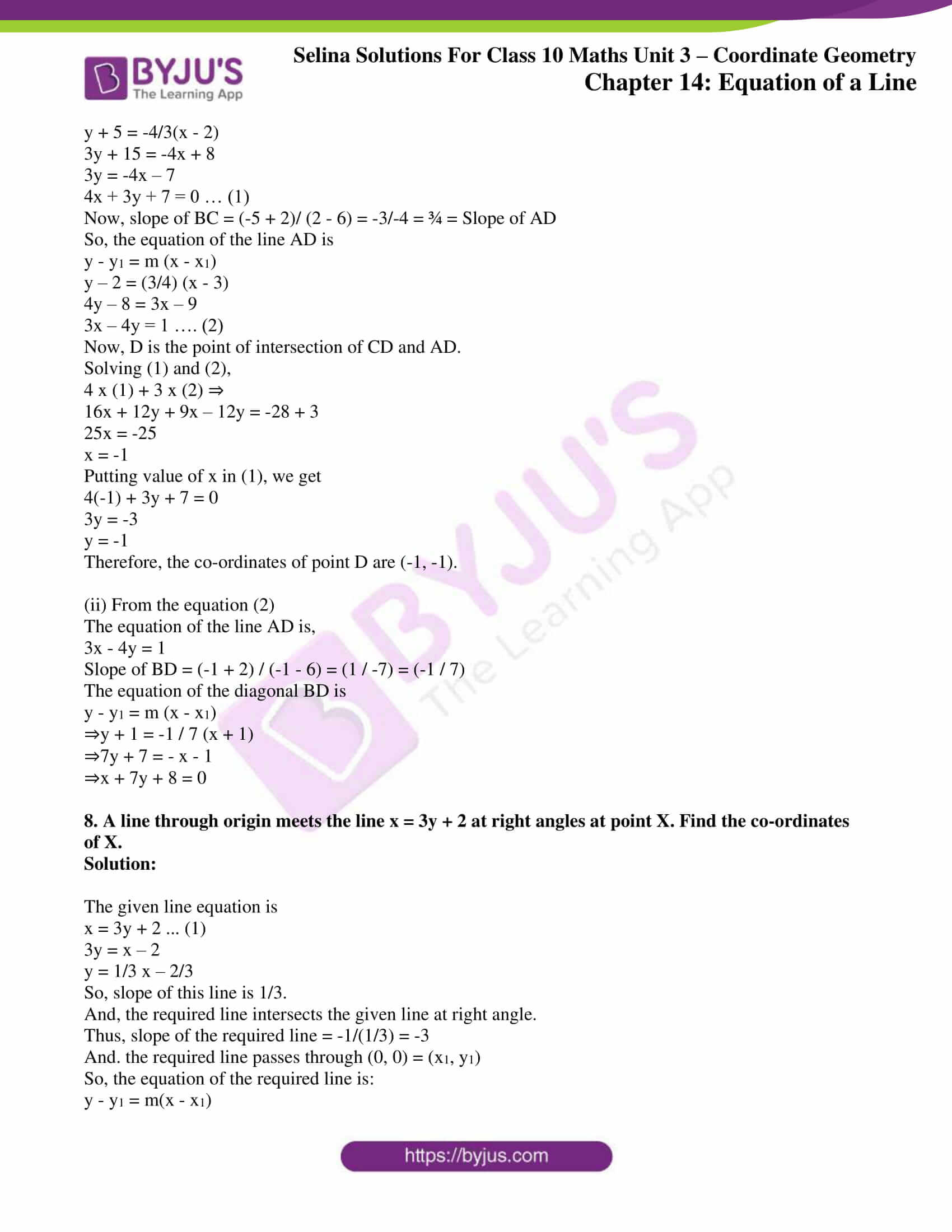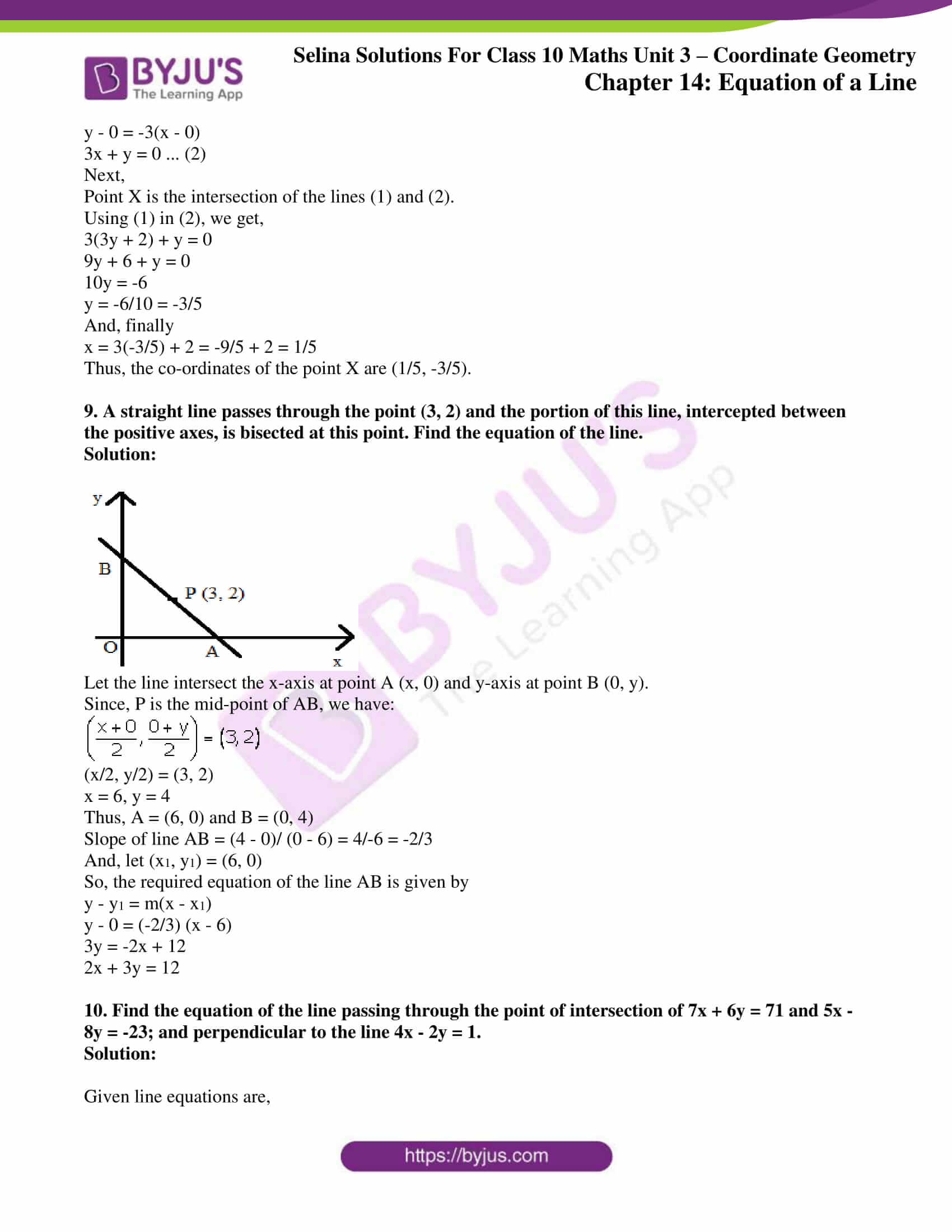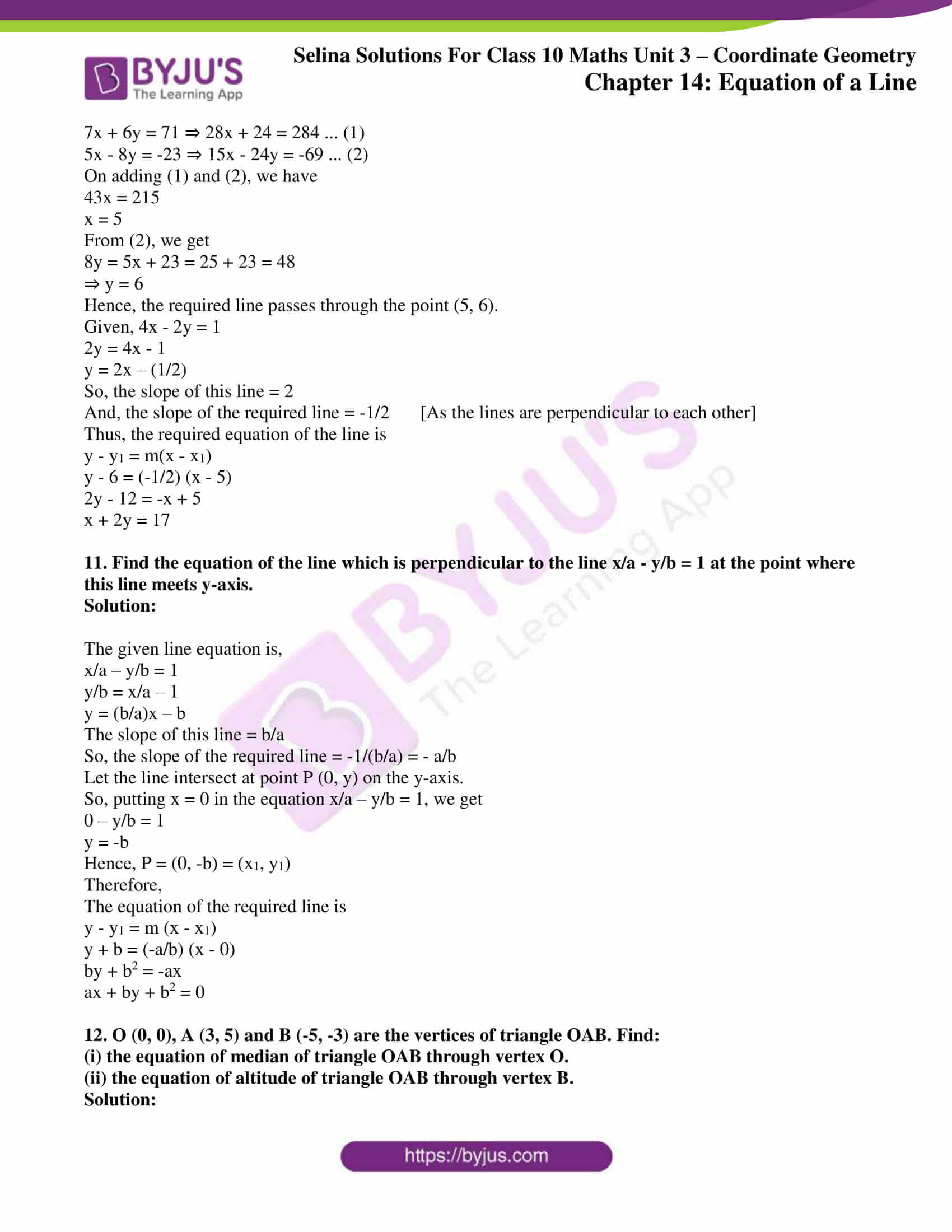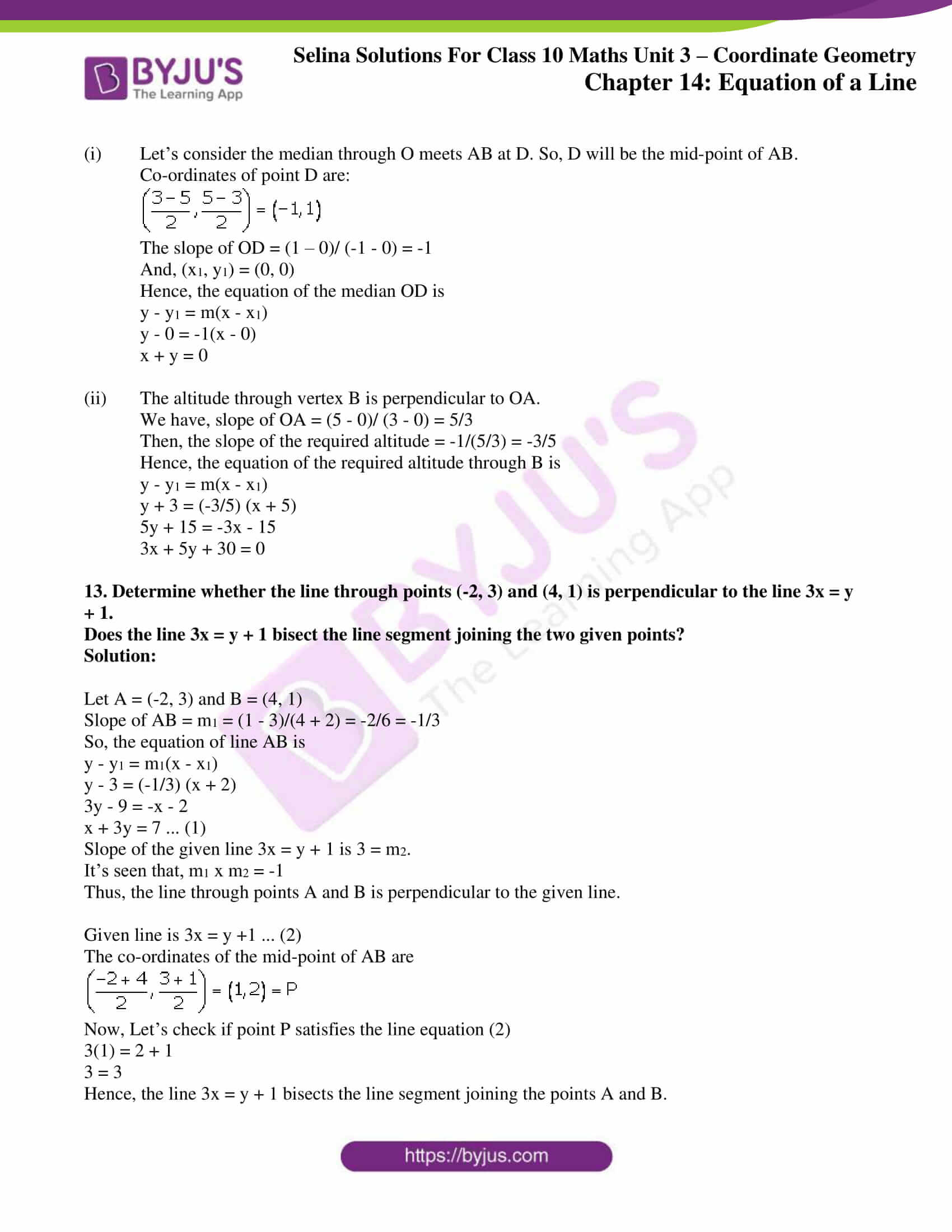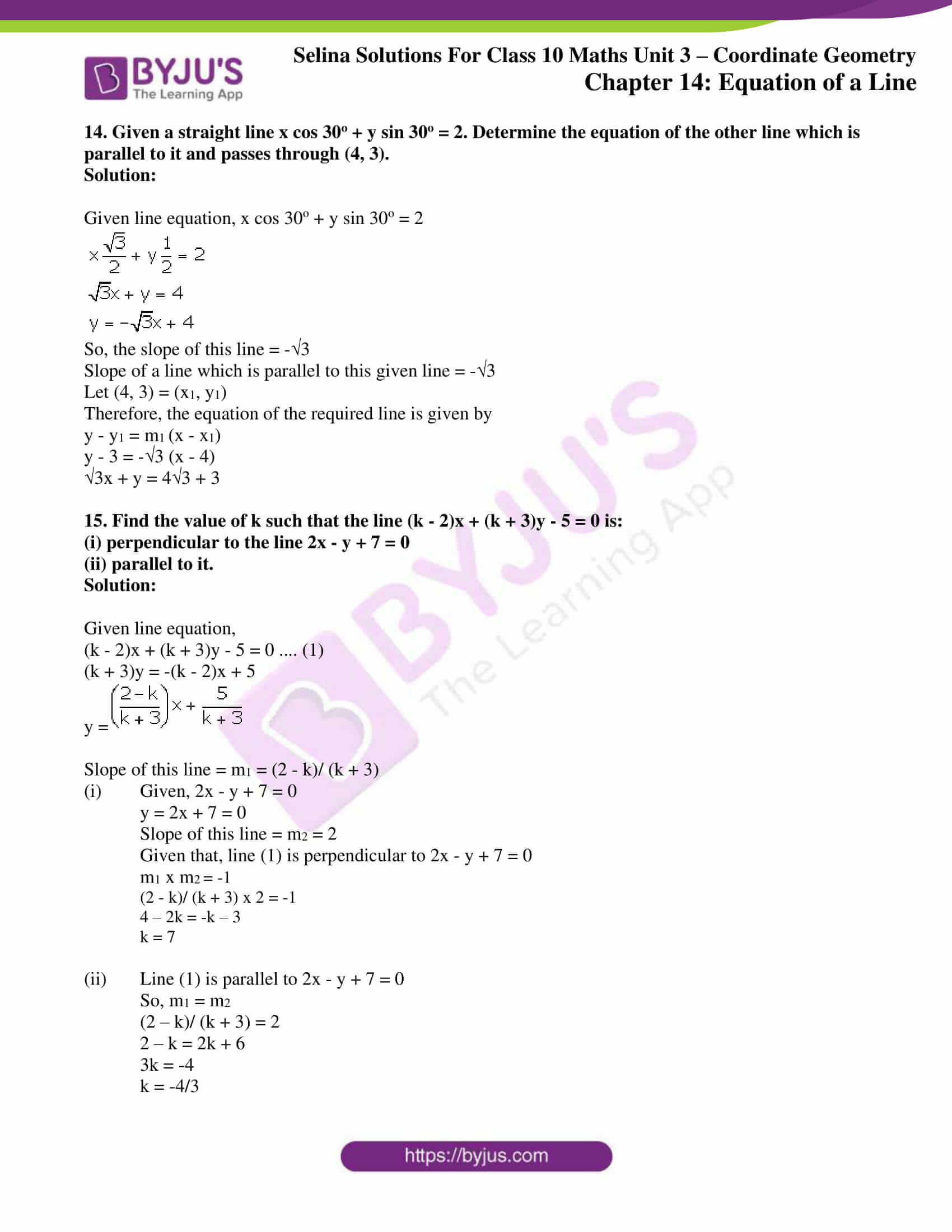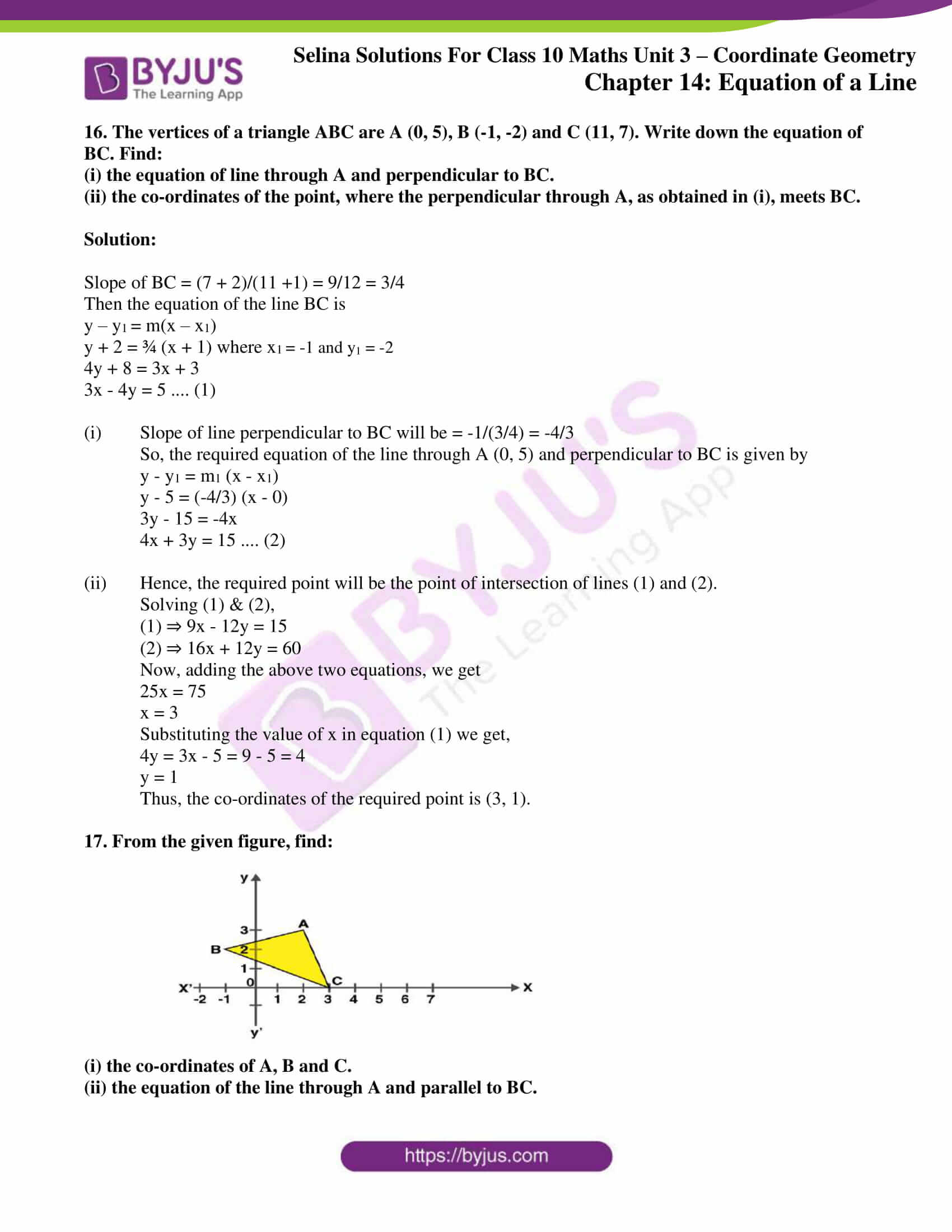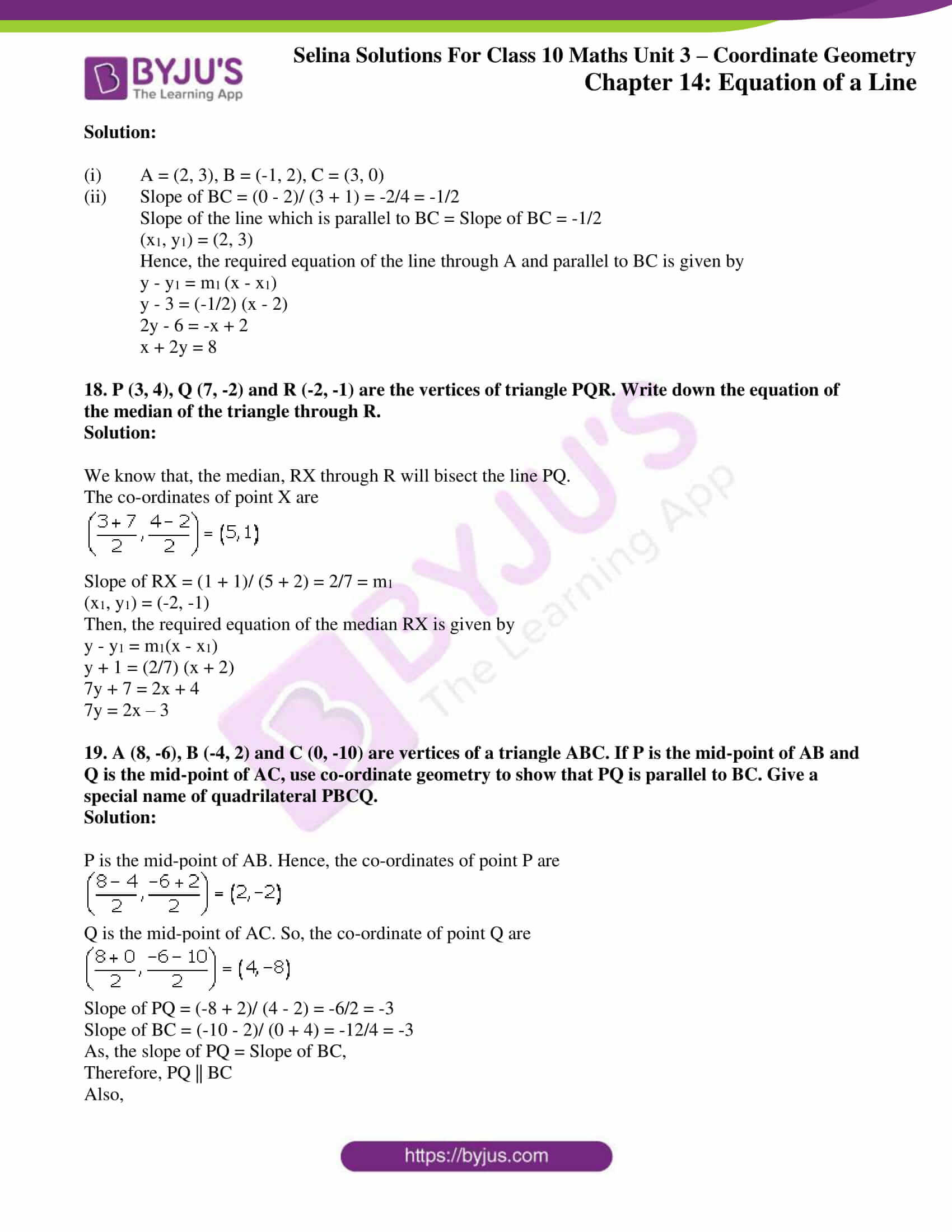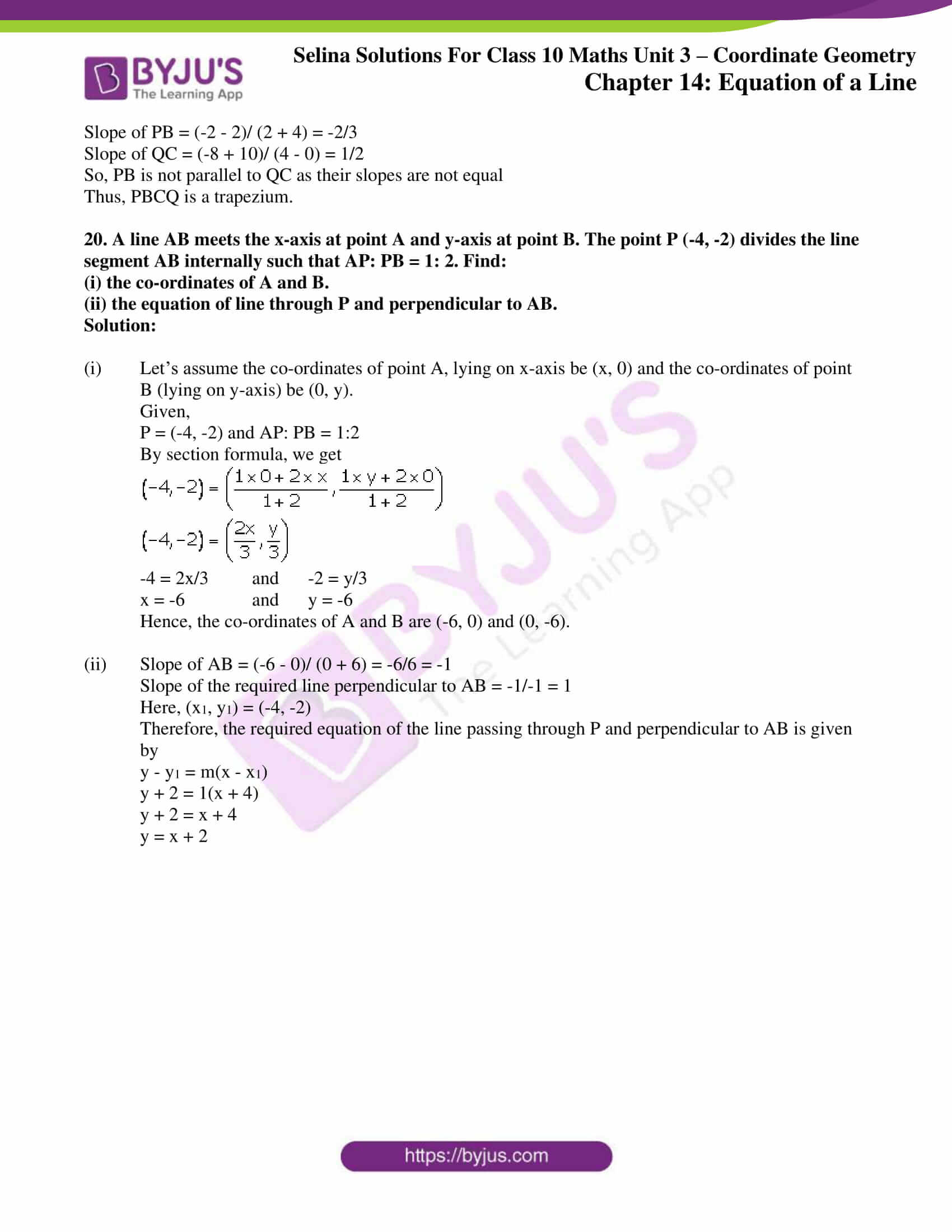## Access Selina Solutions Concise Maths Class 10 Chapter 14 Equation of a Line

### Exercise 14(A) Page No: 185

1. Find, which of the following points lie on the line x – 2y + 5 = 0:

(i) (1, 3) (ii) (0, 5)

(iii) (-5, 0) (iv) (5, 5)

(v) (2, -1.5) (vi) (-2, -1.5)

Solution:

Given line equation is x – 2y + 5 = 0.

(i) On substituting x = 1 and y = 3 in the given line equation, we have

L.H.S. = 1 – 2(3) + 5 = 1 – 6 + 5 = 6 – 6 = 0 = R.H.S.

Hence, the point (1, 3) lies on the given line.

(ii) On substituting x = 0 and y = 5 in the given line equation, we have

L.H.S. = 0 – 2(5) + 5 = -10 + 5 = -5 ≠ R.H.S.

Hence, the point (0, 5) does not lie on the given line.

(iii) On substituting x = -5 and y = 0 in the given line equation, we have

L.H.S. = -5 – 2(0) + 5 = -5 – 0 + 5 = 5 – 5 = 0 = R.H.S.

Hence, the point (-5, 0) lies on the given line.

(iv) On substituting x = 5 and y = 5 in the given line equation, we have

L.H.S. = 5 – 2(5) + 5 = 5 – 10 + 5 = 10 – 10 = 0 = R.H.S.

Hence, the point (5, 5) lies on the given line.

(v) On substituting x = 2 and y = -1.5 in the given line equation, we have

L.H.S. = 2 – 2(-1.5) + 5 = 2 + 3 + 5 = 10 ≠ R.H.S.

Hence, the point (2, -1.5) does not lie on the given line.

(vi) On substituting x = -2 and y = -1.5 in the given line equation, we have

L.H.S. = -2 – 2(-1.5) + 5 = -2 + 3 + 5 = 6 ≠ R.H.S.

Hence, the point (-2, -1.5) does not lie on the given line.

2. State, true or false:

(i) the line x/2 + y/3 = 0  passes through the point (2, 3).

(ii) the line x/2 + y/3 = 0 passes through the point (4, -6).

(iii) the point (8, 7) lies on the line y – 7 = 0.

(iv) the point (-3, 0) lies on the line x + 3 = 0.

(v) if the point (2, a) lies on the line 2x – y = 3, then a = 5.

Solution:

(i) The given line is x/2 + y/3 = 0

Substituting x = 2 and y = 3 in the given equation,

L.H.S = 2/2 + 3/3 = 1 + 1 = 2 ≠ R.H.S

Hence, the given statement is false.

(ii) The given line is x/2 + y/3 = 0

Substituting x = 4 and y = -6 in the given equation,

L.H.S = 4/2 + (-6)/3 = 2 – 2 = R.H.S

Hence, the given statement is true.

(iii) The given line is y – 7 = 0

Substituting y = 7 in the given equation,

L.H.S = y – 7 = 7 – 7 = 0 = R.H.S.

Hence, the given statement is true.

(iv) The given line is x + 3 = 0

Substituting x = -3 in the given equation,

L.H.S. = x + 3 = -3 + 3 = 0 = R.H.S

Hence, the given statement is true.

(v) The point (2, a) lies on the line 2x – y = 3.

Substituting x = 2 and y = a in the given equation, we get

So, 2(2) – a = 3

4 – a = 3

a = 4 – 3 = 1

Hence, the given statement is false.

3. The line given by the equation 2x – y/3 = 7 passes through the point (k, 6); calculate the value of k.

Solution:

Given line equation is 2x – y/3 = 7 passes through the point (k, 6).

So, on substituting x = k and y = 6 in the given equation, we have

2k – 6/3 = 7

6k – 6 = 21

6k = 27

k = 27/6 = 9/2

k = 4.5

4. For what value of k will the point (3, -k) lie on the line 9x + 4y = 3?

Solution:

The given line equation is 9x + 4y = 3.

On putting x = 3 and y = -k, we have

9(3) + 4(-k) = 3

27 – 4k = 3

4k = 27 – 3 = 24

k = 6

5. The line 3x/5 – 2y/3 + 1 = 0 contains the point (m, 2m – 1); calculate the value of m.

Solution:

The equation of the given line is 3x/5 – 2y/3 + 1 = 0

On putting x = m, y = 2m – 1, we have9m – 20m + 10 = -15

-11m = -25

m = 25/11

m =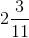6. Does the line 3x – 5y = 6 bisect the join of (5, -2) and (-1, 2)?

Solution:

It’s known that the given line will bisect the join of A (5, -2) and B (-1, 2), if the co-ordinates of the mid-point of AB satisfy the line equation.

The co-ordinates of the mid-point of AB are

(5-1/2, -2+2/2) = (2, 0)

On substituting x = 2 and y = 0 in the given line equation, we have

L.H.S. = 3x – 5y = 3(2) – 5(0) = 6 – 0 = 6 = R.H.S.

Therefore, the line 3x – 5y = 6 bisect the join of (5, -2) and (-1, 2).

### Exercise 14(B) Page No: 190

1. Find the slope of the line whose inclination is:

(i) 0o  (ii) 30o

(iii) 72o 30′ (iv) 46o

Solution:

We know that, the slope of a line is given by the tan of its inclination.

(i) Slope = tan 0o = 0

(ii) Slope = tan 30o = 1/ √3

(iii) Slope = tan 72o 30′ = 3.1716

(iv) Slope = tan 46o = 1.0355

2. Find the inclination of the line whose slope is:

(i) 0 (ii) √3

(iii) 0.7646 (iv) 1.0875

Solution:

(i) Slope = tan θ = 0

⇒ θ = 0o

(ii) Slope = tan θ = √3

⇒ θ = 60o

(iii) Slope = tan θ = 0.7646

⇒ θ = 37o 24′

(iv) Slope = tan θ = 1.0875

⇒ θ = 47o 24′

3. Find the slope of the line passing through the following pairs of points:

(i) (-2, -3) and (1, 2)

(ii) (-4, 0) and origin

(iii) (a, -b) and (b, -a)

Solution:

We know that,

Slope = (y2 – y1)/ (x2 – x1)

(i) Slope = (2 + 3)/ (1 + 2) = 5/3

(ii) Slope = (0 – 0)/ (0 + 4) = 0

(iii) Slope = (-a + b)/ (b – a) = 1

4. Find the slope of the line parallel to AB if:

(i) A = (-2, 4) and B = (0, 6)

(ii) A = (0, -3) and B = (-2, 5)

Solution:

(i) Slope of AB = (6 – 4)/ (0 + 2) = 2/2 = 1

Hence, slope of the line parallel to AB = Slope of AB = 1

(ii) Slope of AB = (5 + 3)/ (-2 – 0) = 8/-2 = -4

Hence, slope of the line parallel to AB = Slope of AB = -4

5. Find the slope of the line perpendicular to AB if:

(i) A = (0, -5) and B = (-2, 4)

(ii) A = (3, -2) and B = (-1, 2)

Solution:

(i) Slope of AB = (4 + 5)/ (-2 – 0) = -9/2

Slope of the line perpendicular to AB = -1/Slope of AB = -1/(-9/2 ) = 2/9

(ii) Slope of AB = (2 + 2)/ (-1 – 3) = 4/-4 = -1

Slope of the line perpendicular to AB = -1/slope of AB = -1/-1 = 1

6. The line passing through (0, 2) and (-3, -1) is parallel to the line passing through (-1, 5) and (4, a). Find a.

Solution:

Slope of the line passing through (0, 2) and (-3, -1) = (-1 – 2)/ (-3 – 0) = -3/-3 = 1

Slope of the line passing through (-1, 5) and (4, a) = (a – 5)/ (4 + 1) = (a -5)/ 5

As, the lines are parallel.

The slopes must be equal.

1 = (a – 5)/ 5

a – 5 = 5

a = 10

7. The line passing through (-4, -2) and (2, -3) is perpendicular to the line passing through (a, 5) and (2, -1). Find a.

Solution:

Slope of the line passing through (-4, -2) and (2, -3) = (-3 + 2)/ (2 + 4) = -1/6

Slope of the line passing through (a, 5) and (2, -1) = (-1 – 5)/ (2 – a) = -6/ (2 –a)

As, the lines are perpendicular we have

-1/6 = -1/(-6/ 2 – a)

-1/6 = (2 – a)/6

2 – a = -1

a = 3

8. Without using the distance formula, show that the points A (4, -2), B (-4, 4) and C (10, 6) are the vertices of a right-angled triangle.

Solution:

Given points are A (4, -2), B (-4, 4) and C (10, 6).

Calculating the slopes, we have

Slope of AB = (4 + 2)/ (-4 – 4) = 6/ -8 = -3/4

Slope of BC = (6 – 4)/ (10 + 4) = 2/ 14 = 1/7

Slope of AC = (6 + 2)/ (10 – 4) = 8/ 6 = 4/3

It’s clearly seen that,

Slope of AB = -1/ Slope of AC

Thus, AB ⊥ AC.

Therefore, the given points are the vertices of a right-angled triangle.

9. Without using the distance formula, show that the points A (4, 5), B (1, 2), C (4, 3) and D (7, 6) are the vertices of a parallelogram.

Solution:

Given points are A (4, 5), B (1, 2), C (4, 3) and D (7, 6).

Slope of AB = (2 – 5)/ (1 – 4) = -3/ -3 = 1

Slope of CD = (6 – 3)/ (7 – 4) = 3/3 = 1

As the slope of AB = slope of CD

So, we can conclude AB || CD

Now,

Slope of BC = (3 – 2)/ (4 – 1) = 1/3

Slope of DA = (6 – 5)/ (7 – 4) = 1/3

As, slope of BC = slope of DA

Hence, we can say BC || DA

Therefore, ABCD is a parallelogram.

10. (-2, 4), (4, 8), (10, 7) and (11, -5) are the vertices of a quadrilateral. Show that the quadrilateral, obtained on joining the mid-points of its sides, is a parallelogram.

Solution:

Let the given points be A (-2, 4), B (4, 8), C (10, 7) and D (11, -5).

And, let P, Q, R and S be the mid-points of AB, BC, CD and DA respectively.

So,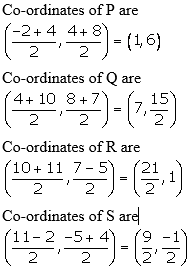As, slope of PQ = Slope of RS, we can say PQ || RS.

Now,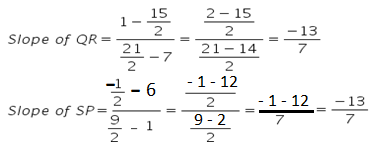As, slope of QR = Slope of SP, we can say QR || SP.

Therefore, PQRS is a parallelogram.

11. Show that the points P (a, b + c), Q (b, c + a) and R (c, a + b) are collinear.

Solution:

We know that,

The points P, Q, R will be collinear if the slopes of PQ and QR are the same.

Calculating for the slopes, we get

Slope of PQ = (c + a – b – c)/ (b – a) = (a – b)/ (b – a) = -1

Slope of QR = (a + b – c – a)/ (c – b) = (b – c)/ (c – b) = -1

Therefore, the points P, Q, and R are collinear.

### Exercise 14(C) Page No: 197

1. Find the equation of a line whose:

y – intercept = 2 and slope = 3.

Solution:

Given,

y – intercept = c = 2 and slope = m = 3.

The line equation is given by: y = mx + c

On substituting the values of c and m, we get

y = 3x + 2

The above is the required line equation.

2. Find the equation of a line whose:

y – intercept = -1 and inclination = 45o.

Solution:

Given,

y – intercept = c = -1 and inclination = 45o.

So, slope = m = tan 45o = 1

Hence, on substituting the values of c and m in the line equation y = mx + c, we get

y = x – 1

The above is the required line equation.

3. Find the equation of the line whose slope is -4/3 and which passes through (-3, 4).

Solution:

Given, slope = -4/3

The line passes through the point (-3, 4) = (x1, y1)

Now, on substituting the values in y – y1 = m(x – x1), we have

y – 4 = -4/3 (x + 3)

3y – 12 = -4x – 12

4x + 3y = 0

Hence, the above is the required line equation.

4. Find the equation of a line which passes through (5, 4) and makes an angle of 60o with the positive direction of the x-axis.

Solution:

The slope of the line, m = tan 60o = √3

And, the line passes through the point (5, 4) = (x1, y1)

Hence, on substituting the values in y – y1 = m(x – x1), we have

y – 4 = √3 (x – 5)

y – 4 = √3x – 5√3

y = √3x + 4 – 5√3, which is the required line equation.

5. Find the equation of the line passing through:

(i) (0, 1) and (1, 2) (ii) (-1, -4) and (3, 0)

Solution:

(i) Let (0, 1) = (x1, y1) and (1, 2) = (x2, y2)

So,

Slope of the line = (2 – 1)/ (1 – 0) = 1

Now,

The required line equation is given by,

y – y1 = m(x – x1)

y – 1 = 1(x – 0)

y – 1 = x

y = x + 1

(ii) Let (-1, -4) = (x1, y1) and (3, 0) = (x2, y2)

So,

Slope of the line = (0 + 4)/ (3 + 1) = 4/4 = 1

The required line equation is given by,

y – y1 = m(x – x1)

y + 4 = 1(x + 1)

y + 4 = x + 1

y = x – 3

6. The co-ordinates of two points P and Q are (2, 6) and (-3, 5) respectively. Find:

(ii) the equation of PQ;

(iii) the co-ordinates of the point where PQ intersects the x-axis.

Solution:

Given,

The co-ordinates of two points P and Q are (2, 6) and (-3, 5) respectively.

(i) Gradient of PQ = (5 – 6)/ (-3 – 2) = -1/-5 = 1/5

(ii) The line equation of PQ is given by

y – y1 = m(x – x1)

y – 6 = 1/5 (x – 2)

5y – 30 = x – 2

5y = x + 28

(iii) Let the line PQ intersect the x-axis at point A (x, 0).

So, on putting y = 0 in the line equation of PQ, we get

0 = x + 28

x = -28

Hence, the co-ordinates of the point where PQ intersects the x-axis are A (-28, 0).

7. The co-ordinates of two points A and B are (-3, 4) and (2, -1). Find:

(i) the equation of AB;

(ii) the co-ordinates of the point where the line AB intersects the y-axis.

Solution:

(i) Given, co-ordinates of two points A and B are (-3, 4) and (2, -1).

Slope = (-1 – 4)/ (2 + 3) = -5/5 = -1

The equation of the line AB is given by:

y – y1 = m(x – x1)

y + 1 = -1(x – 2)

y + 1 = -x + 2

x + y = 1

(ii) Let’s consider the line AB intersect the y-axis at point (0, y).

On putting x = 0 in the line equation, we get

0 + y = 1

y = 1

Thus, the co-ordinates of the point where the line AB intersects the y-axis are (0, 1).

8. The figure given below shows two straight lines AB and CD intersecting each other at point P (3, 4). Find the equation of AB and CD.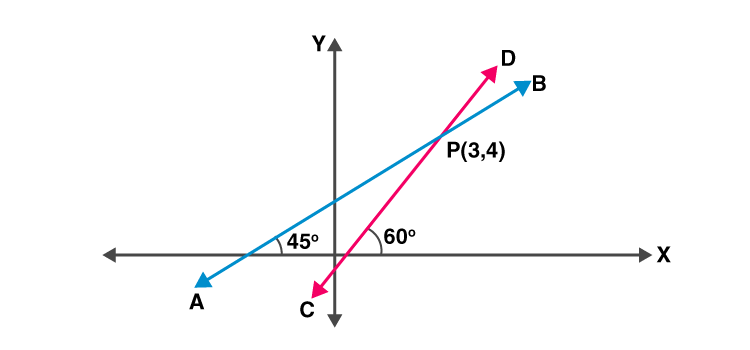Solution:

The slope of line AB = tan 45o = 1

And, the line AB passes through P (3, 4).

Hence, the equation of the line AB is given by

y – y1 = m(x – x1)

y – 4 = 1(x – 3)

y – 4 = x – 3

y = x + 1

The slope of line CD = tan 60o = √3

And, the line CD passes through P (3, 4).

Hence, the equation of the line CD is given by

y – y1 = m(x – x1)

y – 4 = √3 (x – 3)

y – 4 = √3x – 3√3

y = √3x + 4 – 3√3

9. In ΔABC, A = (3, 5), B = (7, 8) and C = (1, -10). Find the equation of the median through A.

Solution:

Given,

Vertices of ΔABC, A = (3, 5), B = (7, 8) and C = (1, -10).

Coordinates of the mid-point D of BC = (x1 + x2) / 2, (y1 + y2) / 2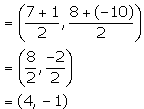The slope of AD = (y2 – y1)/ (x2 – x1)

= (-1 – 5)/ (4 – 3)

= -6/1

= -6

Hence, the equation of the median AD through A is given by

y – y1 = m (x – x1)

y – 5 = -6(x – 3)

y – 5 = -6x + 18

6x + y = 23

10. The following figure shows a parallelogram ABCD whose side AB is parallel to the x-axis,A = 60o and vertex C = (7, 5). Find the equations of BC and CD.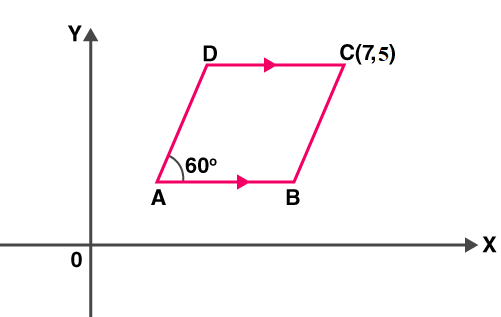Solution:

Given, ∠A = 60o and vertex C = (7, 5)

As, ABCD is a parallelogram, we have

∠A + ∠B = 180o [corresponding angles]

∠B = 180o – 60o = 120o

Slope of BC = tan 120o = tan (90o + 30o) = cot 30o = √3

So, the equation of line BC is given by

y – y1 = m(x – x1)

y – 5 = √3 (x – 7)

y – 5 = √3x – 7√3

y = √3x + 5 – 7√3

As, CD || AB and AB || x-axis

Slope of CD = Slope of AB = 0 [As slope of x-axis is zero]

So, the equation of the line CD is given by

y – y1 = m(x – x1)

y – 5 = 0(x – 7)

y = 5

11. Find the equation of the straight line passing through origin and the point of intersection of the lines x + 2y = 7 and x – y = 4.

Solution:

The given line equations are:

x + 2y = 7 ….(1)

x – y = 4 ….(2)

On solving the above line equations, we can find the point of intersection of the two lines.

So, subtracting (2) from (1), we get

3y = 3

y = 1

Now,

x = 4 + y = 4 + 1 = 5 [From (2)]

It’s given that,

The required line passes through (0, 0) and (5, 1).

The slope of the line = (1 – 0)/ (5 – 0) = 1/5

Hence, the required equation of the line is given by

y – y1 = m(x – x1)

y – 0 = 1/5(x – 0)

5y = x

x – 5y = 0

### Exercise 14(D) Page No: 201

1. Find the slope and y-intercept of the line:

(i) y = 4

(ii) ax – by = 0

(iii) 3x – 4y = 5

Solution:

(i) y = 4

On comparing the given equation with y = mx + c, we get

Slope = m = 0

y – intercept = c = 4

(ii) ax – by = 0 ⇒ by = ax ⇒ y = (a/b)x

On comparing the above equation with y = mx + c, we get

Slope = m = a/b

y – intercept = c = 0

(iii) 3x – 4y = 5 ⇒ 4y = 3x – 5 ⇒ y = ¾ x – 5/4

On comparing the above equation with y = mx + c, we get

Slope = m = 3/4

y-intercept = c = -5/4

2. The equation of a line x – y = 4. Find its slope and y-intercept. Also, find its inclination.

Solution:

Given equation of a line: x – y = 4

⇒ y = x – 4

Comparing the above equation with y = mx + c, we get

Slope = m = 1

y – intercept = c = -4

Let the inclination be θ.

Slope = 1 = tan θ = tan 45o

θ = 45o

3. (i) Is the line 3x + 4y + 7 = 0 perpendicular to the line 28x – 21y + 50 = 0?

(ii) Is the line x – 3y = 4 perpendicular to the line 3x – y = 7?

(iii) Is the line 3x + 2y = 5 parallel to the line x + 2y = 1?

(iv) Determine x so that the slope of the line through (1, 4) and (x, 2) is 2.

Solution:

(i) Given,

3x + 4y + 7 = 0

⇒ 4y = -3x – 7

⇒ y = (-3/4)x – 7/4

Slope of this line = -3/4

And, for

28x – 21y + 50 = 0

⇒ 21y = 28x + 50

⇒ y = (28/21)x + 50/21

⇒ y = (4/3)x + 50/21

Slope of this line = 4/3

As, the product of slopes of the two lines = 4/3 x -3/4 = -1

Therefore, the lines are perpendicular to each other.

(ii) Given,

x – 3y = 4

⇒ 3y = x – 4

⇒ y = (1/3)x – 4/3

So, the slope of this line = 1/3

And, for

3x – y = 7

y = 3x – 7

So, the slope of this line = 3

Now, the slopes of the two lines = 1/3 x 3 = 1 and not equal to -1.

Hence, the lines are not perpendicular to each other.

(iii) Given,

3x + 2y = 5

2y = -3x + 5

y = (-3/2) x + 5/2

So, the slope of this line = -3/2

And, for

x + 2y = 1

2y = -x + 1

y = -1/2x + 1/2

So, the slope of this line = -1/2

The slopes of the two lines are not equal.

Hence, the lines are not parallel to each other.

(iv) Given, the slope of the line through (1, 4) and (x, 2) is 2.

So,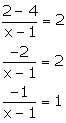-1 = x – 1

x = 0

4. Find the slope of the line which is parallel to:

(i) x + 2y + 3 = 0 (ii) x/2 – y/3 – 1 = 0

Solution:

(i) x + 2y + 3 = 0

2y = -x – 3

y = -1/2x – 3/2

Slope of this line = -1/2

Thus, slope of the line which is parallel to the given line = slope of the given line = -1/2

(ii)  x/2 – y/3 – 1 = 0

y/3 = x/2 – 1

y = (3/2)x – 3

So, the slope of this line = 3/2

Thus, slope of the line which is parallel to the given line = Slope of the given line = 3/2

5. Find the slope of the line which is perpendicular to:

(i) x – y/2 + 3 = 0  (ii) x/3 – 2y = 4

Solution:

(i)  x – y/2 + 3 = 0

y/2 = x + 3

y = 2x + 6

So, the slope of this line = 2

We know that,

Slope of the line which is perpendicular to the given line = -1/(Slope of the given line) = -1/2

(ii)  x/3 – 2y = 4

2y = x/3 – 4

y = x/6 – 2

So, the slope of this line = 1/6

We know that,

Slope of the line which is perpendicular to the given line = -1/(Slope of the given line) = -1/(1/6) = -6

6. (i) Lines 2x – by + 3 = 0 and ax + 3y = 2 are parallel to each other. Find the relation connecting a and b.

(ii) Lines mx + 3y + 7 = 0 and 5x – ny – 3 = 0 are perpendicular to each other. Find the relation connecting m and n.

Solution:

(i) We know that, if two lines are parallel then, the slopes of the two lines must be equal.

For, 2x – by + 3 = 0

by = 2x + 3

y = (2/b)x + 3/b

So, the slope of this line = 2/b

And, ax + 3y = 2

3y = -ax + 2

y = (-a/3)x + 2/3

So, the slope of this line = -a/3

Now, equating the slopes we get

2/b = -a/3

ab = -6

(ii) We know that, if two lines are perpendicular to each other then, the product of their slopes = -1.

For, mx + 3y + 7 = 0

3y = -mx – 7

y = -m/3x – 7/3

Slope of this line = -m/3

And, 5x – ny – 3 = 0

ny = 5x – 3

y = (5/n)x – 3/n

Slope of this line = 5/n

Products of slopes is

(-m/3) x (5/n) = -1

5m = 3n

7. Find the value of p if the lines, whose equations are 2x – y + 5 = 0 and px + 3y = 4 are perpendicular to each other.

Solution:

2x – y + 5 = 0 …. (1)

px + 3y = 4 ….. (2)

y = 2x + 5

Now,

Slope of line = 2

px + 3y = 4

The above equation can be rewritten as

3y = -px + 4

y = (-p/3)x + 4/3

So, the slope of this line = -p/3

For 2 lines to be perpendicular to each other, the product of their slopes must be -1.

So,

(2) x (-p/3) = -1

2p/3 = 1

p = 3/2

8. The equation of a line AB is 2x – 2y + 3 = 0.

(i) Find the slope of the line AB.

(ii) Calculate the angle that the line AB makes with the positive direction of the x-axis.

Solution:

(i) Given, equation of the line

2x – 2y + 3 = 0

2y = 2x + 3

y = x + (3/2)

So, the slope of the line AB = 1

(ii) Required to find the angle of the line AB = θ

We have,

Slope = tan θ = 1

And, tan 45o = 1

Hence, θ = 45o

9. The lines represented by 4x + 3y = 9 and px – 6y + 3 = 0 are parallel. Find the value of p.

Solution:

4x + 3y = 9

3y = -4x + 9

y = (-4/3)x + 3

Slope of this line = -4/3

And,

px – 6y + 3 = 0

6y = px + 3

y = (p/6)x + ½

Slope of this line = p/6

For two lines to be parallel, their slopes must be equal.

-4/3 = p/6

-4 = p/2

p = -8

10. If the lines y = 3x + 7 and 2y + px = 3 are perpendicular to each other, find the value of p.

Solution:

y = 3x + 7

Slope of this line = 3

And,

2y + px = 3

2y = -px + 3

y = (-p/2)x + 3

So, the slope of this line = -p/2

If two lines are perpendicular to each other, then the product of their slopes is -1.

(3) x (-p/2) = -1

3p/2 = 1

p = 2/3

11. The line through A(-2,3) and B(4,b) is perpendicular to the line 2x – 4y =5. Find the value of b.

Solution:

Given,

Points A (-2, 3) and B (4, b)

And, line equation: 2x – 4y = 5

4y = 2x – 5

y = (1/2)x – 5/4

So, the slope of this line = ½

From the question, it’s said that

The line through A and B is perpendicular to above given line.

We know that, when two lines are perpendicular their product of slopes is -1

Hence, the slope of the line through A and B must be -2.

Now,

The slope of the line through A and B is given by,

Slope of AB = (b – 3)/ (4 + 2)

= (b – 3)/6

Thus,

(b – 3)/6 = -2

b – 3 = -12

b = -9

12. Find the equation of the line through (-5, 7) and parallel to:

(i) x-axis (ii) y-axis

Solution:

(i) We know that, the slope of a line parallel to x-axis is 0.

Here, (x1, y1) = (-5, 7) and m = 0

So, the required line equation is

y – y1 = m(x – x1)

y – 7 = 0(x + 5)

y = 7

(ii) We know that, the slope of a line parallel to y-axis is not defined. (tan90o)

So, the given line is parallel to y-axis.

Here, (x1, y1) = (-5, 7)

So, the required equation of the line is

x – x= 0

x + 5 = 0

### Exercise 14(E) Page No: 202

1. Point P divides the line segment joining the points A (8, 0) and B (16, -8) in the ratio 3: 5. Find its co-ordinates of point P.

Also, find the equation of the line through P and parallel to 3x + 5y = 7.

Solution:

Given points, A (8, 0) and B (16, -8)

By section formula, the co-ordinates of the point P which divides AB in the ratio 3: 5 is given by= (11, -3) = (x1, y1)

Given line equation is,

3x + 5y = 7

5y = -3x + 7

y = (-3/5) x + 7/5

So, the slope of this line = -3/5

The line parallel to the line 3x + 5y = 7 will have the same slope,

Hence, the slope of the required line = Slope of the given line = -3/5

Thus,

The equation of the required line is

y – y1 = m(x – x1)

y + 3 = (-3/5)(x – 11)

5y + 15 = -3x + 33

3x + 5y = 18

2. The line segment joining the points A(3, -4) and B (-2, 1) is divided in the ratio 1: 3 at point P in it. Find the co-ordinates of P. Also, find the equation of the line through P and perpendicular to the line 5x – 3y + 4 = 0.

Solution:

Given points, A (3, -4) and B (-2, 1)

By section formula, the co-ordinates of the point P which divides AB in the ratio 1: 3 is given by= (7/4, -11/4) = (x1, y1)

Given line equation is,

5x – 3y + 4 = 0

3y = 5x + 4

y = (5/3) x + 4/3

So, the slope of this line = 5/3

The line perpendicular to the given line will have slope

Slope of the required line = -1/(5/3) = -3/5

Hence,

The equation of the required line is given by

y – y1 = m(x – x1)20y + 55 = -12x + 21

12x + 20y + 34 = 0

6x + 10y + 17 = 0

3. A line 5x + 3y + 15 = 0 meets y-axis at point P. Find the co-ordinates of point P. Find the equation of a line through P and perpendicular to x – 3y + 4 = 0.

Solution:

As the point P lies on y-axis,

Putting x = 0 in the equation 5x + 3y + 15 = 0, we get

5(0) + 3y + 15 = 0

y = -5

Hence, the co-ordinates of the point P are (0, -5).

Given line equation,

x – 3y + 4 = 0

3y = x + 4

y = (1/3)x + 4/3

Slope of this line = 1/3

From the question, the required line equation is perpendicular to the given equation: x – 3y + 4 = 0.

So, the product of their slopes is -1.

Slope of the required line = -1/(1/3) = -3

And,

(x1, y1) = (0, -5)

Therefore,

The required line equation is

y – y1 = m(x – x1)

y + 5 = -3(x – 0)

3x + y + 5 = 0

4. Find the value of k for which the lines kx – 5y + 4 = 0 and 5x – 2y + 5 = 0 are perpendicular to each other.

Solution:

Given,

kx – 5y + 4 = 0

5y = kx + 4

⇒ y = (k/5)x + 4/5

So, the slope of this line = m1 = k/5

And, for 5x – 2y + 5 = 0

⇒ 2y = 5x + 5

y = (5/2) x + 5/2

Slope of this line = m2 = 5/2

As, the lines are perpendicular to each other

m1 x m2 = -1

(k/5) x (5/2) = -1

k = -2

5. A straight line passes through the points P (-1, 4) and Q (5, -2). It intersects the co-ordinate axes at points A and B. M is the mid-point of the segment AB. Find:

(i) the equation of the line.

(ii) the co-ordinates of A and B.

(iii) the co-ordinates of M.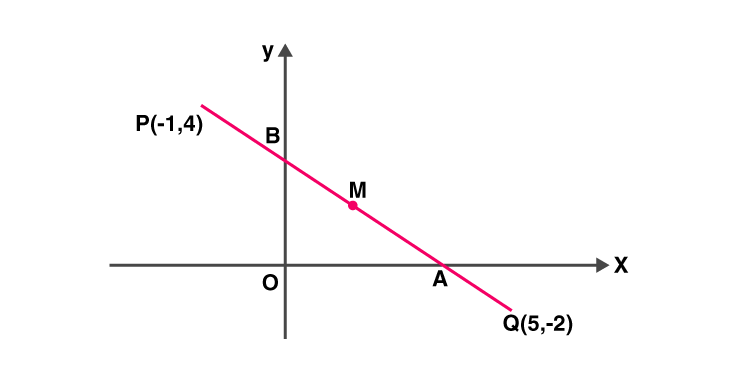Solution:

(i) Given points, P (-1, 4) and Q (5, -2)

Slope of PQ = (-2 – 4)/ (5 + 1) = -6/6 = -1

Equation of the line PQ is given by,

y – y1 = m(x – x1)

y – 4 = -1(x + 1)

y – 4 = -x – 1

x + y = 3

(ii) For point A (on x-axis), y = 0.

So, putting y = 0 in the equation of PQ, we have

x = 3

Hence, the co-ordinates of point A are (3, 0).

For point B (on y-axis), x = 0.

So, putting x = 0 in the equation of PQ, we have

y = 3

Hence, the co-ordinates of point B are (0, 3).

(iii) M is the mid-point of AB.

Thus, the co-ordinates of point M are

(3+0/2, 0+3/2) = (3/2, 3/2)

6. (1, 5) and (-3, -1) are the co-ordinates of vertices A and C respectively of rhombus ABCD. Find the equations of the diagonals AC and BD.

Solution:

Given, A = (1, 5) and C = (-3, -1) of rhombus ABCD.

We know that in a rhombus, diagonals bisect each other at right angle.

Let’s take O to be the point of intersection of the diagonals AC and BD.

Then, the co-ordinates of O are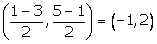Slope of AC = (-1 – 5)/ (-3 – 1) = -6/-4 = 3/2

Then, the equation of the line AC is

y – y1 = m (x – x1)

y – 5 = (3/2) (x – 1)

2y – 10 = 3x – 3

3x – 2y + 7 = 0

Now, the line BD is perpendicular to AC

Slope of BD = -1/(slope of AC) = -2/3

And, (x1, y1) = (-1, 2)

Hence, equation of the line BD is

y – y1 = m (x – x1)

y – 2 = (-2/3) (x + 1)

3y – 6 = -2x – 2

2x + 3y = 4

7. Show that A (3, 2), B (6, -2) and C (2, -5) can be the vertices of a square.

(i) Find the co-ordinates of its fourth vertex D, if ABCD is a square.

(ii) Without using the co-ordinates of vertex D, find the equation of side AD of the square and also the equation of diagonal BD.

Solution:

Given, A (3, 2), B (6, -2) and C (2, -5)

Now, by distance formula

AB = √[(6 – 3)2 + (-2 – 2)2] = √(9 + 16) = 5

BC = √[(2 – 6)2 + (-5 + 2)2] = √(16 + 9) = 5

Thus, AC = BC

Then,

Slope of AB = (-2 – 2)/ (6 – 3) = -4/3

Slope of BC = (-5 + 2)/ (2 – 6) = -3/-4 = ¾

Slope of AB x Slope of BC = -4/3 x ¾ = -1

Hence, AB ⊥ BC

Therefore, A, B, C can be the vertices of a square.

(i) Slope of AB = (-2 – 2)/ (6 – 3) = -4/3 = slope of CD

So, the equation of CD is

y – y1 = m (x – x1)

y + 5 = -4/3(x – 2)

3y + 15 = -4x + 8

3y = -4x – 7

4x + 3y + 7 = 0 … (1)

Now, slope of BC = (-5 + 2)/ (2 – 6) = -3/-4 = ¾ = Slope of AD

So, the equation of the line AD is

y – y1 = m (x – x1)

y – 2 = (3/4) (x – 3)

4y – 8 = 3x – 9

3x – 4y = 1 …. (2)

Now, D is the point of intersection of CD and AD.

Solving (1) and (2),

4 x (1) + 3 x (2) ⇒

16x + 12y + 9x – 12y = -28 + 3

25x = -25

x = -1

Putting value of x in (1), we get

4(-1) + 3y + 7 = 0

3y = -3

y = -1

Therefore, the co-ordinates of point D are (-1, -1).

(ii) From the equation (2)

The equation of the line AD is,

3x – 4y = 1

Slope of BD = (-1 + 2) / (-1 – 6) = (1 / -7) = (-1 / 7)

The equation of the diagonal BD is

y – y1 = m (x – x1)

⇒y + 1 = -1 / 7 (x + 1)

⇒7y + 7 = – x – 1

⇒x + 7y + 8 = 0

8. A line through origin meets the line x = 3y + 2 at right angles at point X. Find the co-ordinates of X.

Solution:

The given line equation is

x = 3y + 2 … (1)

3y = x – 2

y = 1/3 x – 2/3

So, slope of this line is 1/3.

And, the required line intersects the given line at right angle.

Thus, slope of the required line = -1/(1/3) = -3

And. the required line passes through (0, 0) = (x1, y1)

So, the equation of the required line is:

y – y1 = m(x – x1)

y – 0 = -3(x – 0)

3x + y = 0 … (2)

Next,

Point X is the intersection of the lines (1) and (2).

Using (1) in (2), we get,

3(3y + 2) + y = 0

9y + 6 + y = 0

10y = -6

y = -6/10 = -3/5

And, finally

x = 3(-3/5) + 2 = -9/5 + 2 = 1/5

Thus, the co-ordinates of the point X are (1/5, -3/5).

9. A straight line passes through the point (3, 2) and the portion of this line, intercepted between the positive axes, is bisected at this point. Find the equation of the line.

Solution: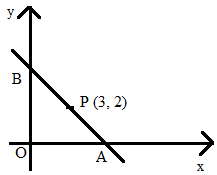Let the line intersect the x-axis at point A (x, 0) and y-axis at point B (0, y).

Since, P is the mid-point of AB, we have:(x/2, y/2) = (3, 2)

x = 6, y = 4

Thus, A = (6, 0) and B = (0, 4)

Slope of line AB = (4 – 0)/ (0 – 6) = 4/-6 = -2/3

And, let (x1, y1) = (6, 0)

So, the required equation of the line AB is given by

y – y1 = m(x – x1)

y – 0 = (-2/3) (x – 6)

3y = -2x + 12

2x + 3y = 12

10. Find the equation of the line passing through the point of intersection of 7x + 6y = 71 and 5x – 8y = -23; and perpendicular to the line 4x – 2y = 1.

Solution:

Given line equations are,

7x + 6y = 71 ⇒ 28x + 24 = 284 … (1)

5x – 8y = -23 ⇒ 15x – 24y = -69 … (2)

On adding (1) and (2), we have

43x = 215

x = 5

From (2), we get

8y = 5x + 23 = 25 + 23 = 48

⇒ y = 6

Hence, the required line passes through the point (5, 6).

Given, 4x – 2y = 1

2y = 4x – 1

y = 2x – (1/2)

So, the slope of this line = 2

And, the slope of the required line = -1/2 [As the lines are perpendicular to each other]

Thus, the required equation of the line is

y – y1 = m(x – x1)

y – 6 = (-1/2) (x – 5)

2y – 12 = -x + 5

x + 2y = 17

11. Find the equation of the line which is perpendicular to the line x/a – y/b = 1 at the point where this line meets y-axis.

Solution:

The given line equation is,

x/a – y/b = 1

y/b = x/a – 1

y = (b/a)x – b

The slope of this line = b/a

So, the slope of the required line = -1/(b/a) = – a/b

Let the line intersect at point P (0, y) on the y-axis.

So, putting x = 0 in the equation x/a – y/b = 1, we get

0 – y/b = 1

y = -b

Hence, P = (0, -b) = (x1, y1)

Therefore,

The equation of the required line is

y – y1 = m (x – x1)

y + b = (-a/b) (x – 0)

by + b2 = -ax

ax + by + b2 = 0

12. O (0, 0), A (3, 5) and B (-5, -3) are the vertices of triangle OAB. Find:

(i) the equation of median of triangle OAB through vertex O.

(ii) the equation of altitude of triangle OAB through vertex B.

Solution:

(i) Let’s consider the median through O meets AB at D. So, D will be the mid-point of AB.

Co-ordinates of point D are: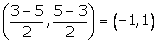The slope of OD = (1 – 0)/ (-1 – 0) = -1

And, (x1, y1) = (0, 0)

Hence, the equation of the median OD is

y – y1 = m(x – x1)

y – 0 = -1(x – 0)

x + y = 0

(ii) The altitude through vertex B is perpendicular to OA.

We have, slope of OA = (5 – 0)/ (3 – 0) = 5/3

Then, the slope of the required altitude = -1/(5/3) = -3/5

Hence, the equation of the required altitude through B is

y – y1 = m(x – x1)

y + 3 = (-3/5) (x + 5)

5y + 15 = -3x – 15

3x + 5y + 30 = 0

13. Determine whether the line through points (-2, 3) and (4, 1) is perpendicular to the line 3x = y + 1.

Does the line 3x = y + 1 bisect the line segment joining the two given points?

Solution:

Let A = (-2, 3) and B = (4, 1)

Slope of AB = m1 = (1 – 3)/(4 + 2) = -2/6 = -1/3

So, the equation of line AB is

y – y1 = m1(x – x1)

y – 3 = (-1/3) (x + 2)

3y – 9 = -x – 2

x + 3y = 7 … (1)

Slope of the given line 3x = y + 1 is 3 = m2.

It’s seen that, m1 x m2 = -1

Thus, the line through points A and B is perpendicular to the given line.

Given line is 3x = y +1 … (2)

The co-ordinates of the mid-point of AB are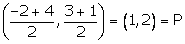Now, Let’s check if point P satisfies the line equation (2)

3(1) = 2 + 1

3 = 3

Hence, the line 3x = y + 1 bisects the line segment joining the points A and B.

14. Given a straight line x cos 30o + y sin 30o = 2. Determine the equation of the other line which is parallel to it and passes through (4, 3).

Solution:

Given line equation, x cos 30o + y sin 30o = 2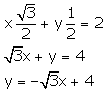So, the slope of this line = -√3

Slope of a line which is parallel to this given line = -√3

Let (4, 3) = (x1, y1)

Therefore, the equation of the required line is given by

y – y1 = m1 (x – x1)

y – 3 = -√3 (x – 4)

√3x + y = 4√3 + 3

15. Find the value of k such that the line (k – 2)x + (k + 3)y – 5 = 0 is:

(i) perpendicular to the line 2x – y + 7 = 0

(ii) parallel to it.

Solution:

Given line equation,

(k – 2)x + (k + 3)y – 5 = 0 …. (1)

(k + 3)y = -(k – 2)x + 5

y =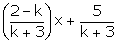Slope of this line = m1 = (2 – k)/ (k + 3)

(i) Given, 2x – y + 7 = 0

y = 2x + 7 = 0

Slope of this line = m2 = 2

Given that, line (1) is perpendicular to 2x – y + 7 = 0

m1 x m2 = -1

(2 – k)/ (k + 3) x 2 = -1

4 – 2k = -k – 3

k = 7

(ii) Line (1) is parallel to 2x – y + 7 = 0

So, m1 = m2

(2 – k)/ (k + 3) = 2

2 – k = 2k + 6

3k = -4

k = -4/3

16. The vertices of a triangle ABC are A (0, 5), B (-1, -2) and C (11, 7). Write down the equation of BC. Find:

(i) the equation of line through A and perpendicular to BC.

(ii) the co-ordinates of the point, where the perpendicular through A, as obtained in (i), meets BC.

Solution:

Slope of BC = (7 + 2)/(11 +1) = 9/12 = 3/4

Then the equation of the line BC is

y – y1 = m(x – x1)

y + 2 = ¾ (x + 1) where x1 = -1 and y1 = -2

4y + 8 = 3x + 3

3x – 4y = 5 …. (1)

(i) Slope of line perpendicular to BC will be = -1/(3/4) = -4/3

So, the required equation of the line through A (0, 5) and perpendicular to BC is given by

y – y1 = m1 (x – x1)

y – 5 = (-4/3) (x – 0)

3y – 15 = -4x

4x + 3y = 15 …. (2)

(ii) Hence, the required point will be the point of intersection of lines (1) and (2).

Solving (1) & (2),

(1) ⇒ 9x – 12y = 15

(2) ⇒ 16x + 12y = 60

Now, adding the above two equations, we get

25x = 75

x = 3

Substituting the value of x in equation (1) we get,

4y = 3x – 5 = 9 – 5 = 4

y = 1

Thus, the co-ordinates of the required point is (3, 1).

17. From the given figure, find: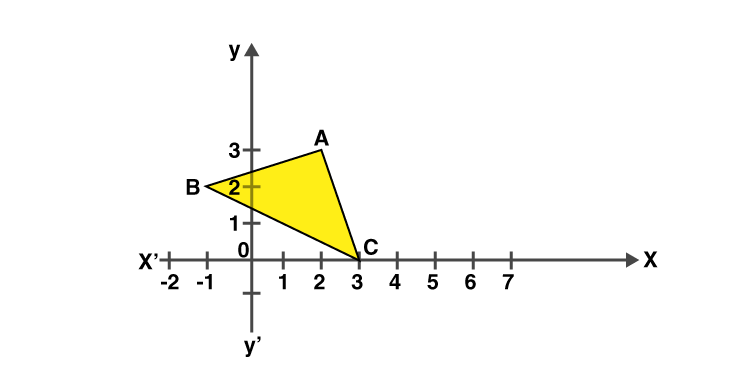(i) the co-ordinates of A, B and C.

(ii) the equation of the line through A and parallel to BC.

Solution:

(i) A = (2, 3), B = (-1, 2), C = (3, 0)

(ii) Slope of BC = (0 – 2)/ (3 + 1) = -2/4 = -1/2

Slope of the line which is parallel to BC = Slope of BC = -1/2

(x1, y1) = (2, 3)

Hence, the required equation of the line through A and parallel to BC is given by

y – y1 = m1 (x – x1)

y – 3 = (-1/2) (x – 2)

2y – 6 = -x + 2

x + 2y = 8

18. P (3, 4), Q (7, -2) and R (-2, -1) are the vertices of triangle PQR. Write down the equation of the median of the triangle through R.

Solution:

We know that, the median, RX through R will bisect the line PQ.

The co-ordinates of point X are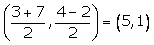Slope of RX = (1 + 1)/ (5 + 2) = 2/7 = m1

(x1, y1) = (-2, -1)

Then, the required equation of the median RX is given by

y – y1 = m1(x – x1)

y + 1 = (2/7) (x + 2)

7y + 7 = 2x + 4

7y = 2x – 3

19. A (8, -6), B (-4, 2) and C (0, -10) are vertices of a triangle ABC. If P is the mid-point of AB and Q is the mid-point of AC, use co-ordinate geometry to show that PQ is parallel to BC. Give a special name of quadrilateral PBCQ.

Solution:

P is the mid-point of AB. Hence, the co-ordinates of point P are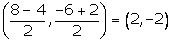Q is the mid-point of AC. So, the co-ordinate of point Q are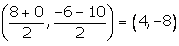Slope of PQ = (-8 + 2)/ (4 – 2) = -6/2 = -3

Slope of BC = (-10 – 2)/ (0 + 4) = -12/4 = -3

As, the slope of PQ = Slope of BC,

Therefore, PQ || BC

Also,

Slope of PB = (-2 – 2)/ (2 + 4) = -2/3

Slope of QC = (-8 + 10)/ (4 – 0) = 1/2

So, PB is not parallel to QC as their slopes are not equal

Thus, PBCQ is a trapezium.

20. A line AB meets the x-axis at point A and y-axis at point B. The point P (-4, -2) divides the line segment AB internally such that AP: PB = 1: 2. Find:

(i) the co-ordinates of A and B.

(ii) the equation of line through P and perpendicular to AB.

Solution:

(i) Let’s assume the co-ordinates of point A, lying on x-axis be (x, 0) and the co-ordinates of point B (lying on y-axis) be (0, y).

Given,

P = (-4, -2) and AP: PB = 1:2

By section formula, we get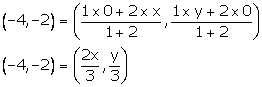-4 = 2x/3 and -2 = y/3

x = -6 and y = -6

Hence, the co-ordinates of A and B are (-6, 0) and (0, -6).

(ii) Slope of AB = (-6 – 0)/ (0 + 6) = -6/6 = -1

Slope of the required line perpendicular to AB = -1/-1 = 1

Here, (x1, y1) = (-4, -2)

Therefore, the required equation of the line passing through P and perpendicular to AB is given by

y – y1 = m(x – x1)

y + 2 = 1(x + 4)

y + 2 = x + 4

y = x + 2

### Exercises of Selina Solutions Concise Maths Class 10 Chapter 14 Equation of a Line

Exercise 14(A) Solutions

Exercise 14(B) Solutions

Exercise 14(C) Solutions

Exercise 14(D) Solutions

Exercise 14(E) Solutions